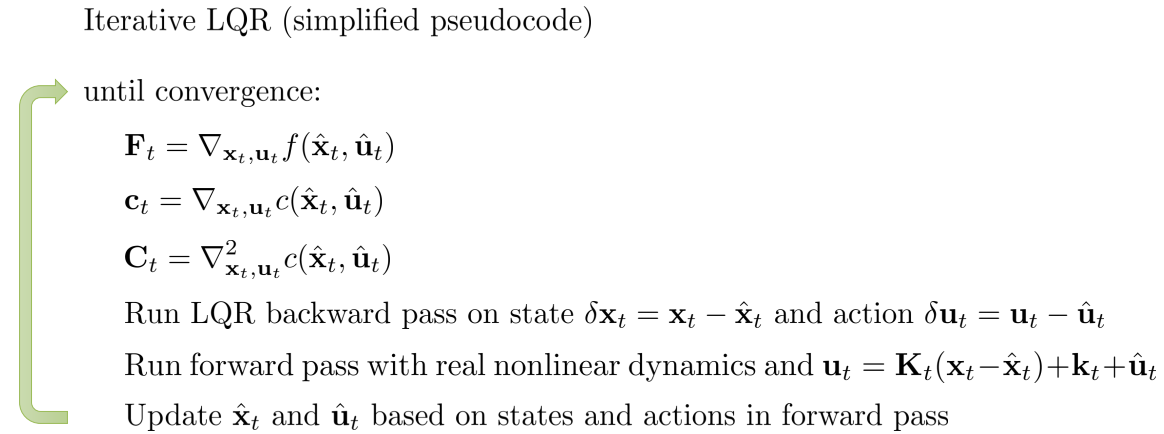# Reinforcement Learning学习笔记（上）

## 入坑指南

Posted by HC on May 3, 2018

# 0. Dynamic System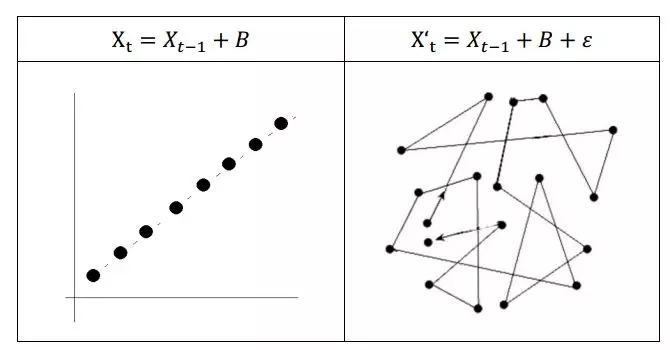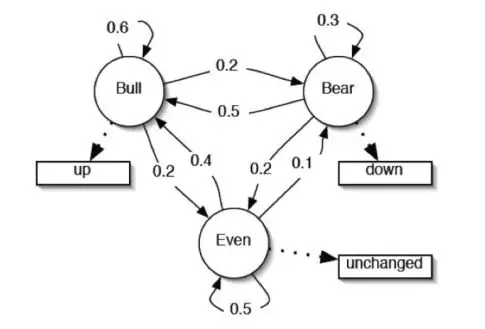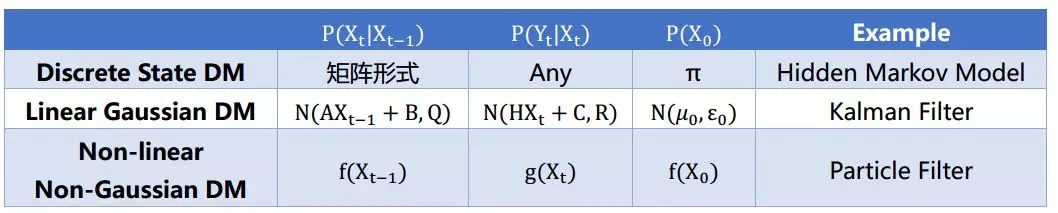1. 离散状态的动态系统的例子就是隐马尔科夫模型（HMM）。它由一个State Transition Matrix $P(x’\mid x)$Measurement Matrix $P(y\mid x)$和一个Initial State π组成。注意：HMM 的状态X必须是离散值，而不是连续值，否则 HMM 的状态转移矩阵就是无穷大的。而对Measurement Y没有做要求。
2. 线性高斯动态系统非线性非高斯动态系统的状态是连续的，线性高斯的State Transition Matrix和Measurement Matrix对应服从高斯分布，而非线性非高斯则可以是任意的。线性高斯动态系统的例子是Kalman Filter，而非线性非高斯的例子是Partical Filter

1. HMM上的常用的操作，一是 uncertainty measurement，已知模型参数和一个观测序列，求它产生的概率，二是 parameter learning，顾名思义就是去对λ = {A, B, π}进行求解。还有一种是做 variable Inference: 在 B 发生的条件下，A 发生的概率 $P(B\mid A)$

2. 卡尔曼滤波是线性动态系统的一种，它的图模型也和 HMM 一样，只是状态取值是连续值，并且它的噪声服从零均值高斯分布，transition probability 和 measurement probability 都服从高斯分布。卡尔曼滤波上的常用操作是 filtering, 给定 T = 1 到 t 时间的观测值，希望对时间 T=t 时的状态进行求解，目标为$P(X_{t}\mid Y_{1}, Y_{2}, … , Y_{t})$。这个filtering是不断的 predict （求解$P(X_{t}\mid Y_{1}, Y_{2}, … , Y_{t-1})$）和不断 update（求解$P(X_{t}\mid Y_{1}, Y_{2}, … , Y_{t})$） 的过程。如下图：3. Particle filter 可以看错是一种MC Sampling的算法。具体来说他是建立在Sequential Importance Sampling（SIS）基础之上的。这个SIS算法是为了解决 Importance Sampling 的不能很好处理高维数据的问题，SIS 旨在对每个样本一维一维的进行采样。particle filter 是通过使用了SIS 进行求解的模型，其中 filtering 的分布由采样数据的位置和其权重来刻画

4. Particle filter 与 Kalman filter 的区别在于，后者认为 measurement 和 transfer probability服从高斯分布，从而 filtering 的分布也服从高斯，他建立起的是 t 时刻和 t+1 时刻 filtering 高斯分布的参数联系；而 particle filter 可以适用于任何分布，所以求解麻烦，转而求助于 SIS 的方法，以当前时刻采样出来的 particle 的位置和权重来表示当前时刻的 filtering 分布。

# 1. Markov Process

MP是一个状态序列满足马尔科夫条件独立性假设（即给定当前时刻的状态，下一时刻的状态与历史时刻的状态保持独立）的随机过程 $$P(S_{t+1}\mid S_t) = P(S_{t+1}\mid S_1, S_2, ..., S_t)$$ MP由两个部分组成：有限状态集合$S$和状态转移矩阵$P$，$P_{ss’}=P(S_{t+1}=s’\mid S_{t}=s)$。以下图为例，这是一个学生上课的MP图。学生需要听过三节课之后才能通过（pass）本门课，圆圈和方块表示状态，其中方块表示终止状态（只进不出），边上的数字表示状态转移概率。在第一节课的时候，同学可能会开小差，去刷facebook，并且一旦开始刷facebook，就会有极大的概率（0.9）一直刷下去。同理，也可能上课睡觉，或者课后去酒吧（pub），喝得烂醉之后重新听课。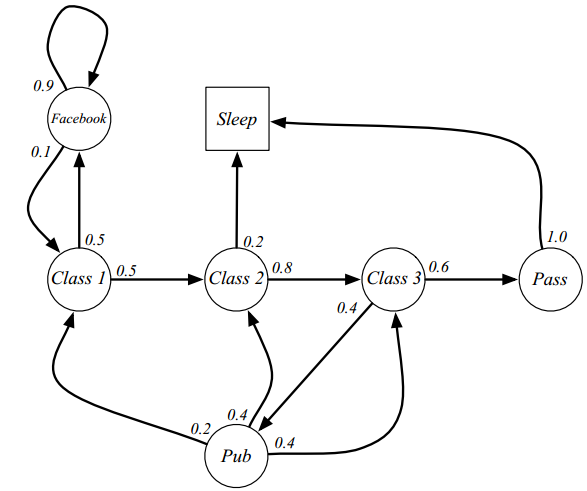1. C1 C2 C3 Pass Sleep
2. C1 FB FB C1 C2 Sleep

3. C1 C2 C3 Pub C2 C3 Pass Sleep
4. ……..

# 2. Markov Reward Process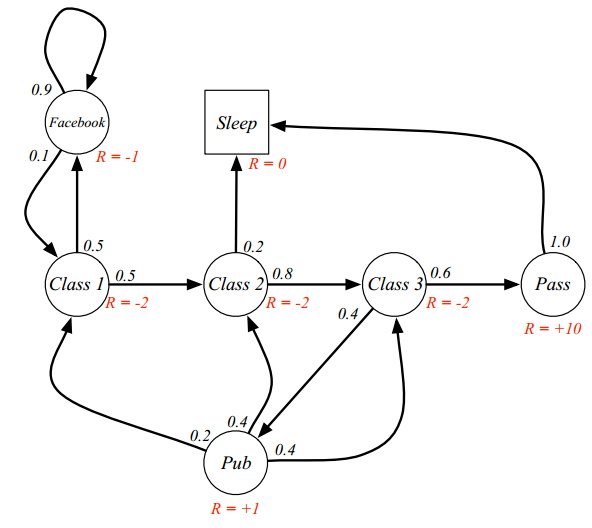1. 有限状态集S
2. 状态转移P，$P_{ss’}=P(S_{t+1}=s’\mid S_{t}=s)$
3. Reward函数 R，$R_{s}=E[R_{t+1}\mid S_{t}=s]$。注意状态s上的Reward是一个期望值
4. 折扣因子（Discount Factor）$\gamma \in [0, 1]$。

1. 避免无穷值（万一MRP没有终止状态，或者MRP中有环）。
2. 保留对未来的不确定性。（注意，$R_{s}$是一个期望值，$R_{t}$不是）
3. 其他解释：现在的钱比未来更值钱

1. C1 C2 C3 Pass Sleep ：G1=-2 -2* (1/2)-2* (1/4)+10* (1/8)
2. C1 FB FB C1 C2 Sleep ：G1=-2-1* (1/2) -1 * (1/4) -2* (1/8) -2 *(1/16)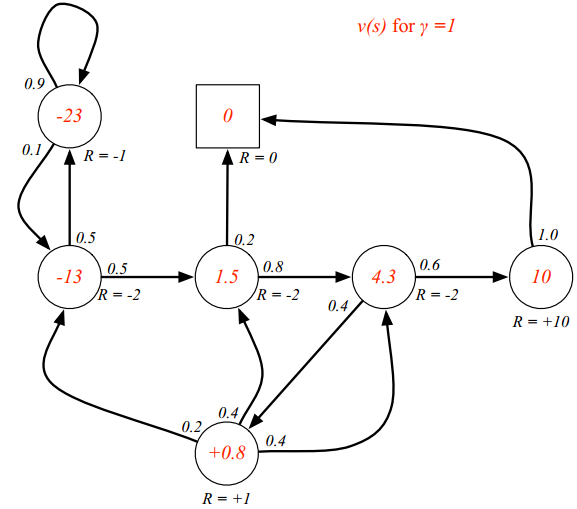1. immediate reward $R_{t+1}$
2. 下一个状态的discounted $\gamma v(S_{t+1})$

$v(s)=E[G_t\mid S_t=s]\\ =E[R_{t+1}+\gamma R_{t+2} + \gamma^2R_{t+3}+...\mid S_t=s]\\ =E[R_{t+1} + \gamma G_{t+1}\mid S_t=s]=E[R_{t+1} + \gamma v(S_{t+1})\mid S_t=s]$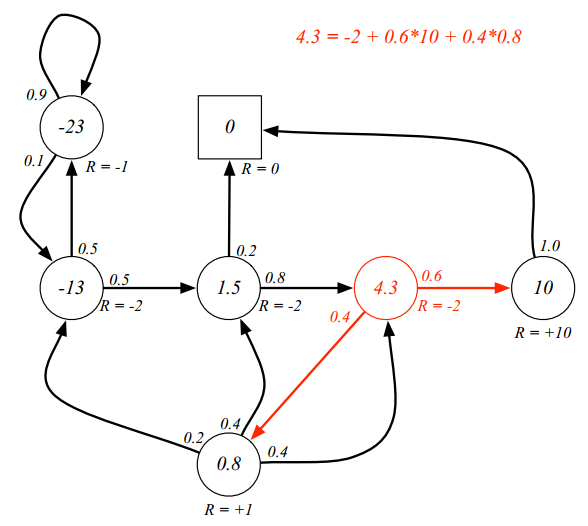$v=R+\gamma Pv = \begin{bmatrix} v(1) \\ ... \\ v(n) \end{bmatrix} = \begin{bmatrix} R_1 \\ ... \\ R_n \end{bmatrix} + \gamma \begin{bmatrix} P_{11} & ... & P_{1n}\\ ... &... & ...\\ P_{n1} & ... & P_{nn} \end{bmatrix} \begin{bmatrix} v(1) \\ ... \\ v(n) \end{bmatrix}$

$v=(I-\gamma P)^{-1}R$

# 3. Markov Decision Process

1. 有限状态集合S
2. 有限动作（决策）集合A
3. 状态转移P，$P_{ss’}^a=P[S_{t+1}=s’\mid S_{t}=s, A_{t}=a]$
4. 奖励函数R，$R_{s}^a=E[R_{t+1}\mid S_{t}=s,A_{t}=a]$
5. 折扣因子$\lambda \in [0,1]$

MRP中，处于某个状态的事物可以做出一个决策动作。根据所处的状态以及所做的决策动作，该事物会获得一个reward，然后转移到新的状态。下图中就是大家熟悉而又喜爱的学生状态图，图中红色的字表示action。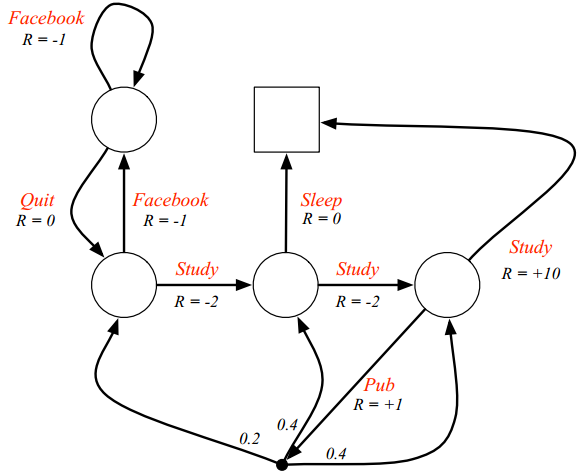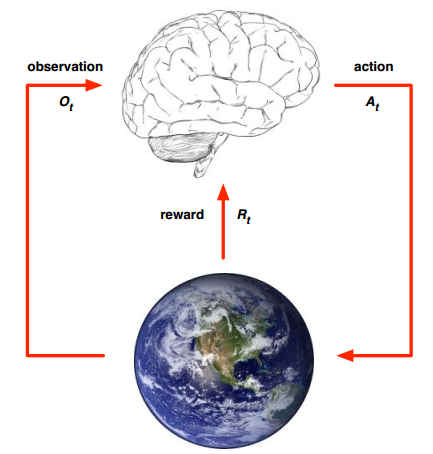$S_t=f(H_t)\\ \mbox{history：}H_t=O_1, R_1, A_1, ..., A_{t-1}, O_{t}, R_{t}$

$q_\pi(s,a)=E_\pi[G_t\mid S_t=s,A_t=a]$

$v_\pi(s)=E_\pi[R_{t+1}+\gamma v_\pi(S_{t+1})\mid S_t=s]\\ q_\pi(s,a)=E_\pi[R_{t+1}+\gamma q_\pi(S_{t+1},A_{t+1})\mid S_t=s, A_t=a]$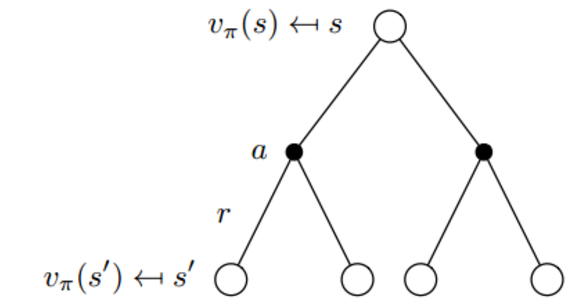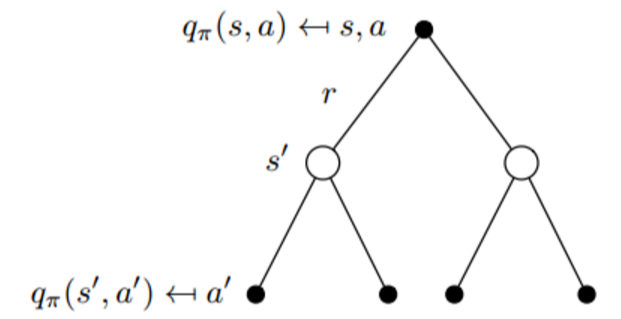$v_\pi(s) = E_\pi[R_{t+1} + \gamma v_{\pi}(S_{t+1}) \mid S_t=s] = \sum_{a\in A} \pi(a\mid s) q_{\pi}(s,a)\\ q_\pi(s,a) = E_\pi[R_{t+1} + \gamma q_{\pi}(S_{t+1}, A_{t+1}) \mid S_t=s, A_t=a] = R_s^a + \gamma \sum_{s' \in S}P^a_{ss'} v_{\pi}(s')\\ v_\pi(s) = \sum_{a\in A} \pi(a\mid s) \left( R_s^a + \gamma \sum_{s' \in S}P^a_{ss'} v_{\pi}(s')\right)$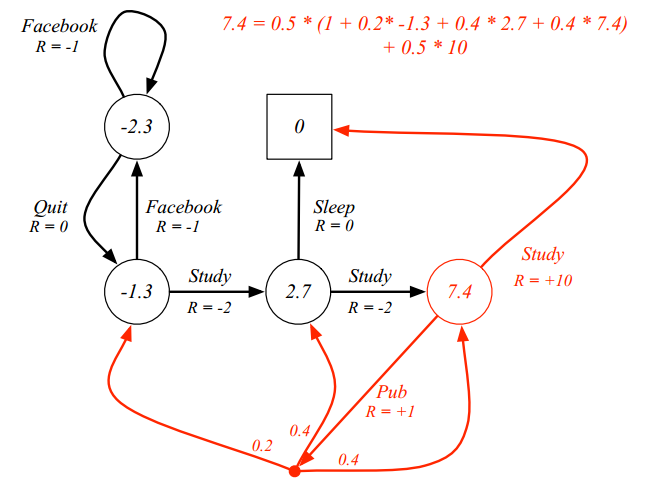$v_*(s)=\max_\pi v_\pi(s)\\ q_*(s,a)=\max_\pi q_\pi(s,a)$

$\pi^*(a\mid s) = \left\{\begin{matrix} 1 \mbox{ if }a=\arg\max_{a\in A}q_*(s,a) \\ 0 \mbox{ otherwise} \end{matrix}\right.$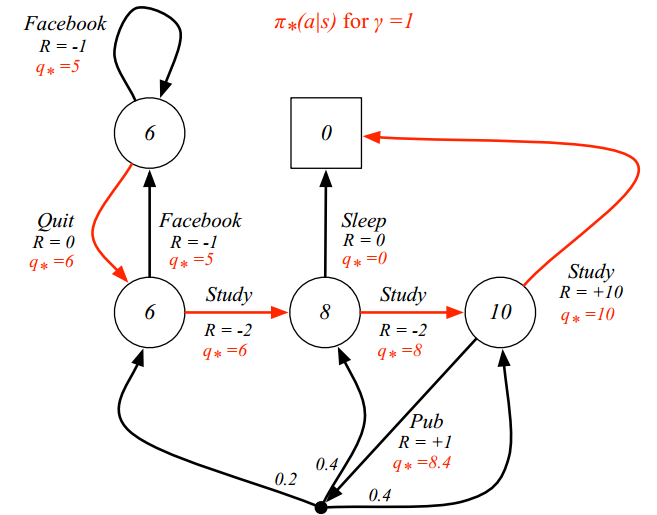$v_*(s)=\max_a q_*(s,a)\\ q_*(s,a) = R_s^a + \gamma \sum_{s' \in S} P_{ss'}^a v_*(s')$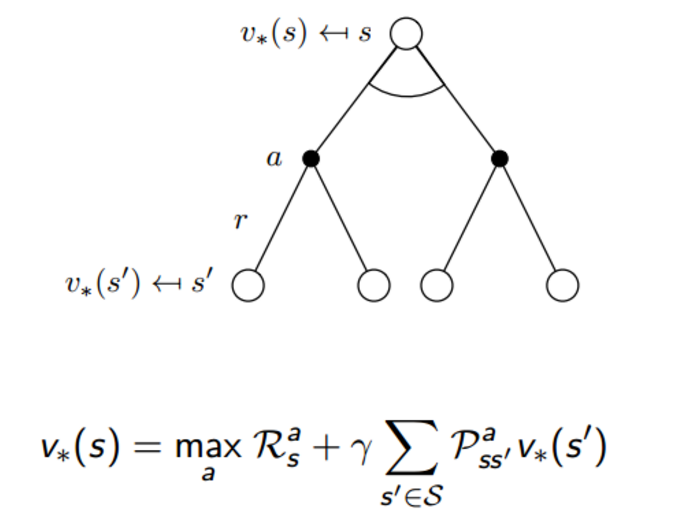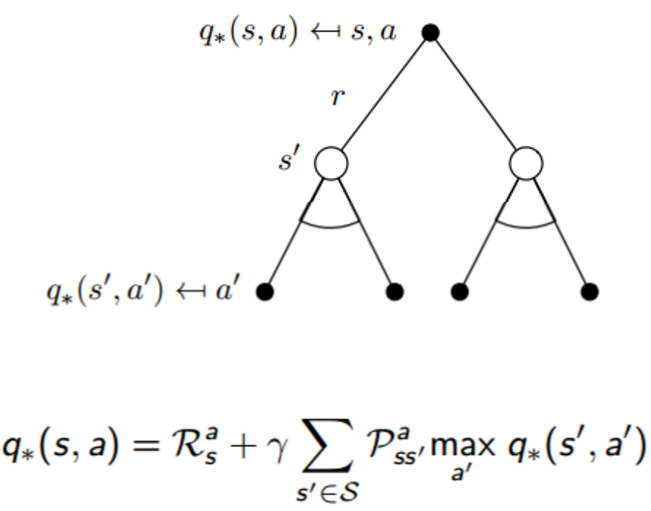# 4. Reinforcement Learning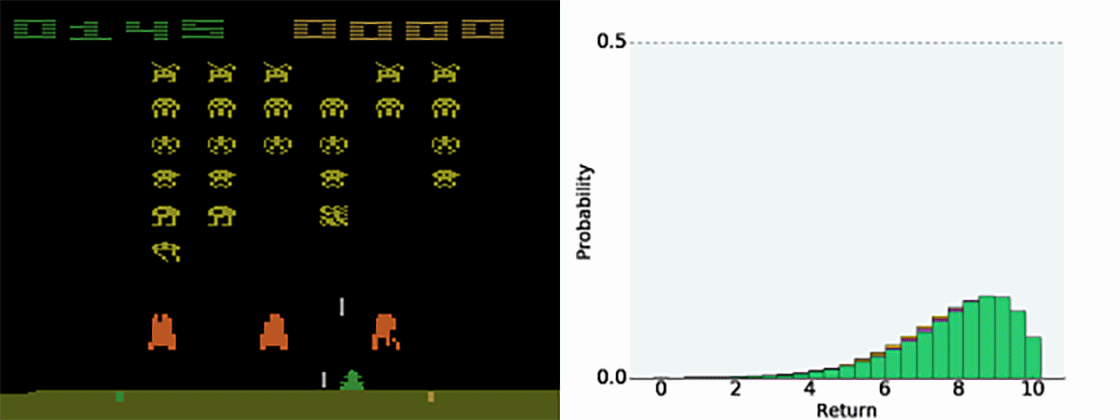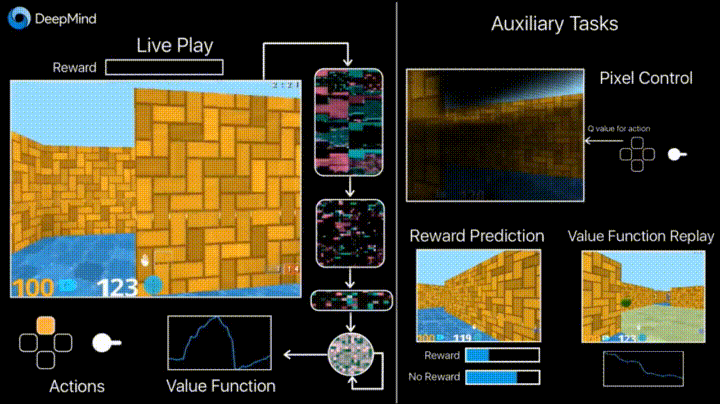1. RL的反馈是可以有时延的。实际情况下，并不是做一个动作之后一定会收到一个真正的reward，比如有的游戏要玩到结束之后，才会收到reward，胜利+1，失败-1。监督学习的标签则不会这样
2. RL处理的是时序episode，监督学习是iid data
3. agent的决策动作是会影响下一步的进展的。

$\max_\pi E_\pi \left[ \sum_{t}r(s_t,a_t) \right]$

1. Prediction：给定policy，估计未来, 找出value function
2. Control：在所有可能的policy中，最好的value function是啥？哪个是最好的policy？

1. Model-Free RL：不知道model（一般来说是指不知道状态转移以及reward function）
2. Model-Based RL：知道model

1. value-based RL：在没有明确的policy下，去找value function
2. policy-based RL：在没有value function的条件下，去找policy
3. Actor-critic RL：用当前的policy来估计value ，并用它来提升policy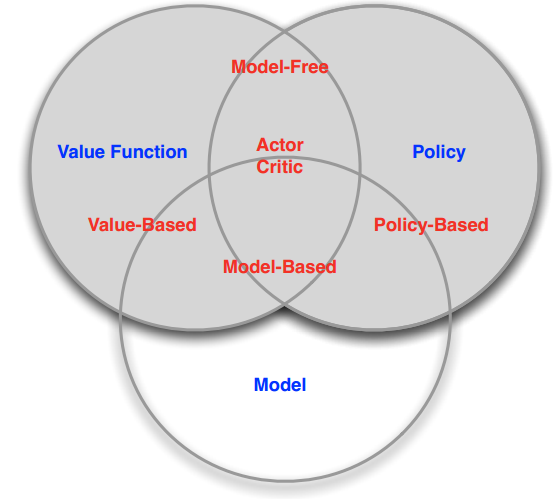RL的过程可以看做是agent和environment之间的游戏，是state和action之间的转换游戏，分别对应了transition和policy。他们可以用下面的图例过程表示。这里的参数$\theta$是用来parameterize策略的（比如使用神经网络来刻画policy，那么$\theta$就是神经网络的参数）。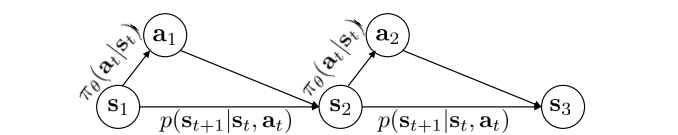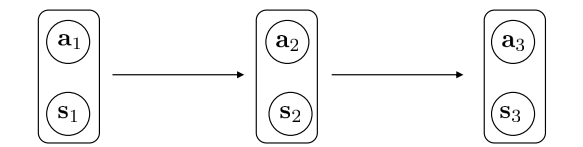$p((s_{t+1},a_{t+1})\mid (s_{t},a_t))=p(s_{t+1}\mid s_t,a_t)\pi_\theta(a_{t+1}\mid s_{t+1})$

$p_\theta(s_1,a_1,...s_T,a_T)=p(s_1) \prod_{t=1}^T \pi_\theta(a_t\mid s_t)p(s_{t+1}\mid s_t,a_t)$

# 5. Policy Gredients

$\theta^*=\arg\max_\theta E_{\tau \sim \pi_\theta(\tau)} \left[ \sum_{t=1} \gamma^{t-1} r(s_t,a_t) \right]=\arg\max_\theta \int \pi_\theta(\tau) r(\tau) d\tau\\ \pi_\theta(\tau)=p_\theta(s_1,a_1,...,s_T,a_T)=p(s_1) \prod_{t=1}^T \pi_\theta(a_t\mid s_t)p(s_{t+1}\mid s_t,a_t)\\ r(\tau)=\sum_{t=1} \gamma^{t-1}r(s_t,a_t)$

$\bigtriangledown_\theta J(\theta)=\int \bigtriangledown_\theta\pi_\theta(\tau) r(\tau) d\tau$

$\bigtriangledown_\theta\pi_\theta(\tau)=\pi_\theta(\tau) \frac{\bigtriangledown_\theta\pi_\theta(\tau)}{\pi_\theta(\tau)}=\pi_\theta(\tau)\bigtriangledown_\theta log \pi_\theta(\tau)$

$\pi_\theta(a\mid s)= \frac{e^{\phi(s) ^T \theta }}{\sum_a e^{\phi(s) ^T \theta}} \\ \bigtriangledown_\theta log \pi_\theta(a\mid s)=\phi(s) - \sum_{a' \in A} \pi_\theta(a'\mid s) \phi(s)$

$\pi_\theta(a\mid s)=N(\phi(s) ^T \theta;\Sigma)\\ log\pi_\theta(a\mid s)=-\frac{1}{2} \mid \mid \phi(s) ^T \theta-a\mid \mid _\Sigma^2 +const\\ \bigtriangledown_\theta log \pi_\theta(a\mid s)=-\frac{1}{2}\Sigma^{-1}(\phi(s) ^T \theta-a) \phi(s)$

$\bigtriangledown_\theta J(\theta)=\int \pi_\theta(\tau)\bigtriangledown_\theta log \pi_\theta(\tau) r(\tau) d\tau=E_{\tau \sim \pi_\theta(\tau)} [\bigtriangledown_\theta log \pi_\theta(\tau) r(\tau)]\\ =E_{\tau \sim \pi_\theta(\tau)} \left[ \left(\sum_{t=1}^T \bigtriangledown_\theta log \pi_\theta(a_t\mid s_t) \right) \left( \sum_{t=1}^T \gamma^{t-1} r(s_t,a_t)\right) \right]$

$\bigtriangledown_\theta J(\theta) \approx \frac{1}{N} \sum^N_{i=1} \left( \left(\sum_{t=1}^T \bigtriangledown_\theta log \pi_\theta(a_{i,t}\mid s_{i,t}) \right) \left( \sum_{t=1}^T \gamma^{t-1} r(s_{i,t},a_{i,t})\right) \right)$

$\bigtriangledown_\theta J(\theta) \approx \frac{1}{N} \sum^N_{i=1} \left( \left(\sum_{t=1}^T \bigtriangledown_\theta log \pi_\theta(a_{i,t}\mid s_{i,t}) \right) \left( \sum_{t=1}^T \gamma^{t-1} r(s_{i,t},a_{i,t})\right) \right)\\ =\frac{1}{N} \sum^N_{i=1} \bigtriangledown_\theta log \pi_\theta(\tau_i) r(\tau_i)\\ \bigtriangledown_\theta J_{ML}(\theta) \approx \frac{1}{N} \sum^N_{i=1} \left(\sum_{t=1}^T \bigtriangledown_\theta log \pi_\theta(a_{i,t}\mid s_{i,t}) \right)=\frac{1}{N} \sum^N_{i=1} \bigtriangledown_\theta log \pi_\theta(\tau_i)$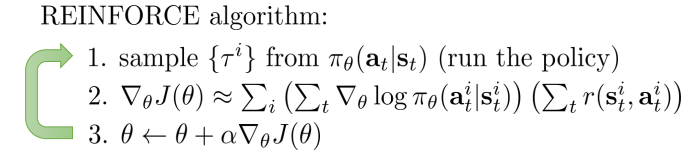1. Causality, reward to go：今天做的动作，不会影响昨天发生的事
2. Baselines：选一个比平均动作更好的动作

Causality, Reward to go

$\bigtriangledown_\theta J(\theta) \approx \frac{1}{N} \sum^N_{i=1} \left( \left(\sum_{t=1}^T \bigtriangledown_\theta log \pi_\theta(a_{i,t}\mid s_{i,t}) \right) \left( \sum_{t‘=t}^T \gamma^{t'-t} r(s_{i,t'},a_{i,t'})\right) \right)$

Baselines

Baseline想做的是，与其直接最大化每个episode的累计reward，不如最大化每个episode reward 与 平均reward的差值，使得episode能做得比平均值更好。对于比平均做得差的，那就降低他的出现概率。

$\bigtriangledown_\theta J(\theta) \approx \frac{1}{N} \sum^N_{i=1} \bigtriangledown_\theta log \pi_\theta(\tau_i)[ r(\tau_i) - b]\\ b=f(r(\tau))$

$E[ \bigtriangledown_\theta log \pi_\theta(\tau) b]=\int \pi_\theta(\tau) \bigtriangledown_\theta log\pi_\theta(\tau) b d\tau=\int \bigtriangledown_\theta \pi_\theta(\tau) bd\tau=b\bigtriangledown_\theta \int \pi_\theta(\tau) d\tau=0$

$Var=E_{\tau \sim \pi_\theta(\tau)}[\left( \bigtriangledown_\theta log \pi_\theta(\tau)[ r(\tau) - b] \right)^2] - E_{\tau \sim \pi_\theta(\tau)}[\bigtriangledown_\theta log \pi_\theta(\tau)[ r(\tau) - b] ]^2$

$b=\frac{E\left[(\bigtriangledown_\theta log \pi_\theta(\tau))^2 r(\tau)\right]}{E\left[(\bigtriangledown_\theta log \pi_\theta(\tau))^2\right]}\\ b=\frac{1}{N}\sum_{i=1}^N r(\tau_i)$

$E_{x \sim p(x)}[f(x)]=\int p(x)f(x)dx=\int q(x)\frac{p(x)}{q(x)}f(x)dx=E_{x \sim q(x)}[\frac{p(x)}{q(x)}f(x)]$

$J(\theta) = E_{\tau \sim \pi'(\tau)} [\frac{\pi_\theta(\tau)}{\pi'(\tau)}r(\tau)]\\ \frac{\pi_\theta(\tau)}{\pi'(\tau)}=\frac{p(s_1) \prod_{t=1}^T \pi_\theta(a_t\mid s_t)p(s_{t+1}\mid s_t,a_t)}{p(s_1) \prod_{t=1}^T \pi'(a_t\mid s_t)p(s_{t+1}\mid s_t,a_t)} = \prod_{t=1}^T \frac{\pi_\theta(a_t\mid s_t)}{\pi'(a_t\mid s_t)}$

$\bigtriangledown_\theta J(\theta) = E_{\tau \sim \pi'(\tau)} [\frac{\pi_\theta(\tau)}{\pi'(\tau)} \bigtriangledown_\theta log \pi_\theta(\tau) r(\tau)]\\ =E_{\tau \sim \pi'(\tau)} \left[ \left(\prod_{t=1}^T \frac{\pi_\theta(a_t\mid s_t)}{\pi'(a_t\mid s_t)}\right)\left(\sum_{t=1}^T \bigtriangledown_\theta log \pi_\theta(a_{i,t}\mid s_{i,t}) \right) \left( \sum_{t‘=1}^T \gamma^{t'-1} r(s_{i,t'},a_{i,t'})\right)\right] \\ =E_{\tau \sim \pi'(\tau)} \left[ \sum_{t=1}^T \bigtriangledown_\theta log \pi_\theta(a_{i,t}\mid s_{i,t}) \left(\prod_{t'=1}^t \frac{\pi_\theta(a_t'\mid s_t')}{\pi'(a_t'\mid s_t')}\right) \left( \sum_{t‘=t}^T \gamma^{t'-t} r(s_{i,t'},a_{i,t'})\right)\right]$

### 5.3 Other Policy Gradients Methods

$\frac{\partial J(\theta)}{\partial \theta_k} \approx \frac{J(\theta+\epsilon u_k)-J(\theta)}{\epsilon}$

$\bigtriangledown_\theta J(\theta)=E_{\tau \sim \pi_\theta(\tau)} [\bigtriangledown_\theta log \pi_\theta(\tau) r(\tau)]$

$\bigtriangledown_\theta J(\theta)=E_{\tau \sim \pi_\theta(\tau)} [\bigtriangledown_\theta log \pi_\theta(\tau) r(\tau)] \rightarrow \mbox{ REINFORCE algorithm} \\ \bigtriangledown_\theta J(\theta)=E_{\tau \sim \pi_\theta(\tau)} [\bigtriangledown_\theta log \pi_\theta(\tau) Q_w(\tau)] \rightarrow\mbox{ Q Actor-Critic algorithm}\\ \bigtriangledown_\theta J(\theta)=E_{\tau \sim \pi_\theta(\tau)} [\bigtriangledown_\theta log \pi_\theta(\tau) A_w(\tau)]\rightarrow\mbox{ Advantage Actor-Critic algorithm}\\ \bigtriangledown_\theta J(\theta)=E_{\tau \sim \pi_\theta(\tau)} [\bigtriangledown_\theta log \pi_\theta(\tau) \delta ]\rightarrow\mbox{ TD Actor-Critic algorithm}\\ \bigtriangledown_\theta J(\theta)=E_{\tau \sim \pi_\theta(\tau)} [\bigtriangledown_\theta log \pi_\theta(\tau) \delta e]\rightarrow\mbox{ TD(lambda) Actor-Critic algorithm}\\ ...$

# 6. Actor-Critic Algorithms

## 6.1 Reduce variance by actor-critic algorithm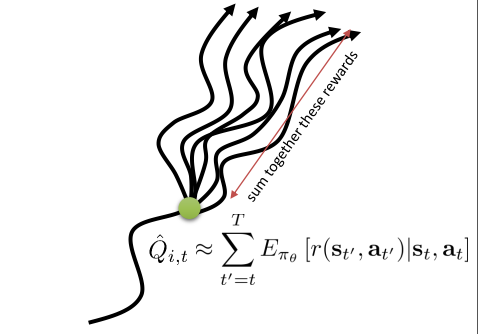$\bigtriangledown_\theta J(\theta) \approx \frac{1}{N} \sum^N_{i=1} \sum_{t=1}^T \bigtriangledown_\theta log \pi_\theta(a_{i,t}\mid s_{i,t}) Q(s_{i,t},a_{i,t})$

$V(s_{t})=E_{a_t \sim \pi_\theta(a_t \mid s_t)}[Q(s_{t},a_{t})]$

$Q(s_t,a_t)=r(s_t,a_t)+V(S_{t+1})=r(s_t,a_t)+E_{s_{t+1} \sim P(s_{t+1}\mid s_{t}, a_t)}[V(s_{t+1})]$

$Q(s_t,a_t) = \sum_{t'=t} \gamma^{t'-t} r(s_{t'},a_{t'})\\ A_{MC}(s_t,a_t) \approx \sum_{t'=t} \gamma^{t'-t} r(s_{t'},a_{t'}) - V(s_t)$

$Q(s_t,a_t) \approx r(s_t,a_t)+V(s_{t+1})\\ A_{Bootstrap}(s_t,a_t) \approx r(s_t,a_t)+V(s_{t+1}) - V(s_t)$

1. Critic：用于更新Value function，判断当前policy的好坏
2. Actor：接收critic的信息，用于更新policy（update policy in direction suggested by critic）

## 6.2 Improve policy gradient by critic

### 6.2.1 Policy evaluation with known MDPs

policy evaluation是建立上Bellman Equation之上的。用上一时刻的value function来做更新

$v_{k+1}(s)=\sum_{a \in A} \pi(a\mid s) \left(R_s^a + \gamma \sum_{s' \in S}P^a_{ss'} v_k(s') \right)$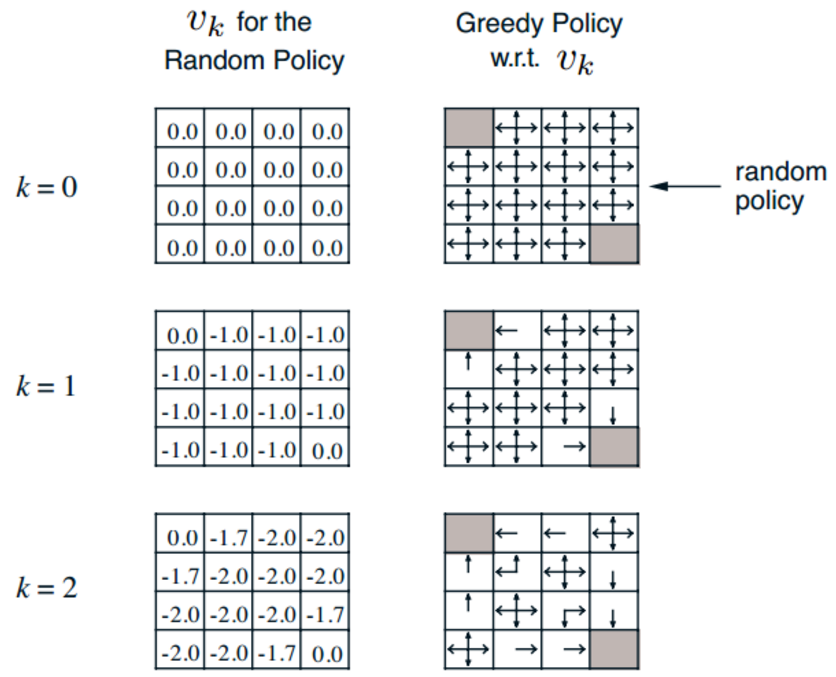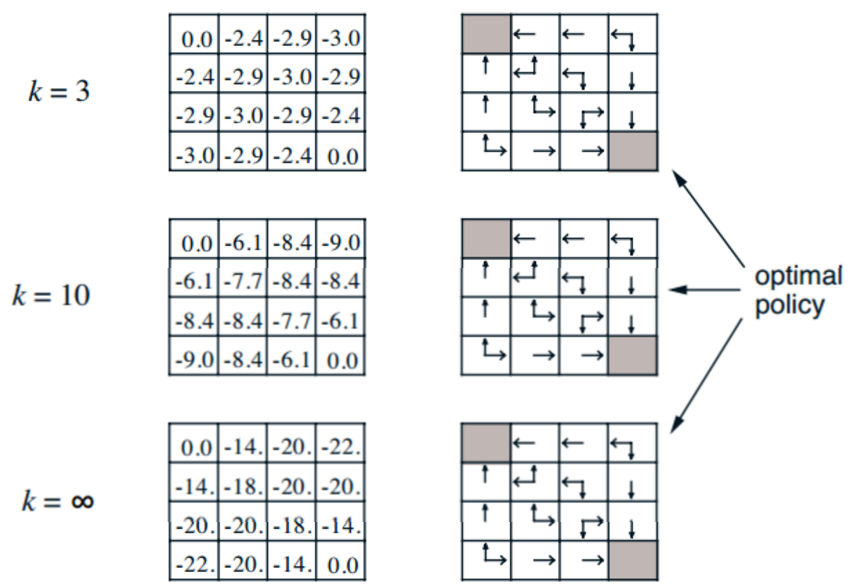### 6.2.2 Policy evaluation with unknown MDPs

#### 6.2.2.1 Monte-Carlo Learning

MC走的就是采样的路，用样本来近似求解，比如用 样本均值来近似期望等等。所以，MC的方式是用过样本的平均return来近似State-value function的。但是，MC方法需要对整个episode进行采样，必须要得到整条episode之后，才能做后续的操作（这里隐藏暗示了每一个episode都会terminate），所以MC方法一般是off-line update的。

MC方法一般有两种

1. First-visit Monte-Carlo Policy Evaluation
2. Every-visit Monte-Carlo Policy Evaluation

1. 采用好多个episodes
2. 遍历每一个episode
1. 如果s在该episode中的第t时刻被第一次访问到了
1. $N(s) += 1$
2. $S(s) += G_{t}$
3. 计算$V(s)=\frac{S(s)}{N(s)}$

$u_k=\frac{1}{k} \sum_{j=1}^k x_j=\frac{1}{k} \left( x_k + \sum_{j=1}^{k-1}x_j \right) = \frac{1}{k} (x_k + (k-1)u_{k-1})=u_{k-1}+\frac{1}{k}(x_k-u_{k-1})$

$N(S_t) += 1 \\ V(S_t) \leftarrow V(S_t) + \frac{1}{N(S_{t})} (G_t -V(S_t))$

$N(S_t) += 1 \\ V(S_t) \leftarrow V(S_t) +\alpha (G_t -V(S_t))$

#### 6.2.2.2 Temporal-Difference Learning (TD Learning)

TD Learning不必从完整的episode中学习，反而是采用bootstrapping（引导）的方式进行online的更新。具体来说，TD采用以下的更新方式。其中R是immediate reward

$V(S_t) \leftarrow V(S_t) +\alpha (R_{t+1} + \gamma V(S_{t+1}) -V(S_t))$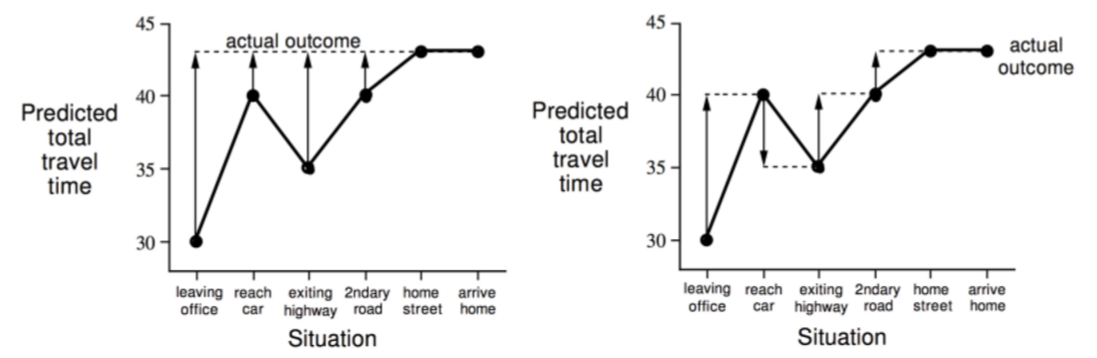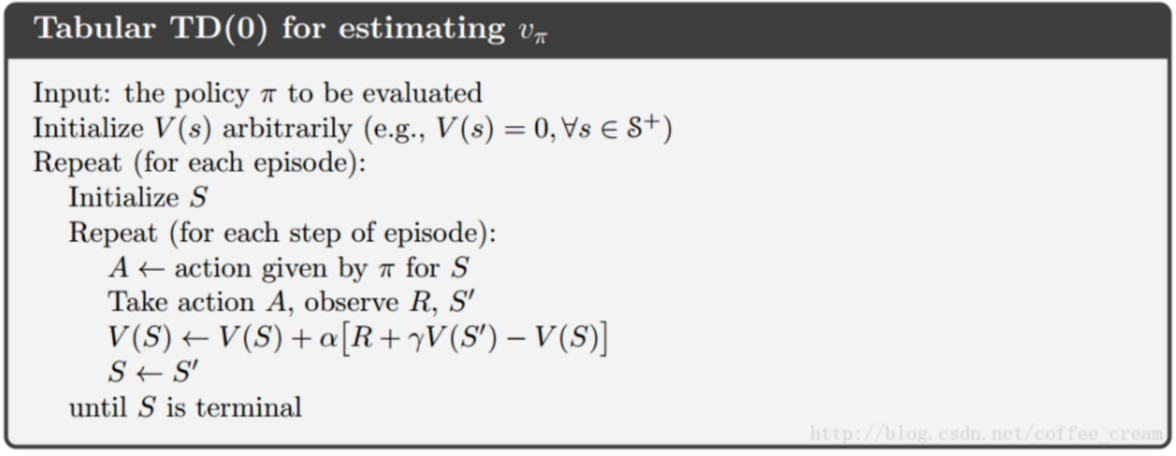1. MC采用了整个episode的return来做更新，TD采用TD target，可以在线更新
2. MC方式不能用于没有终止状态的MDP，TD可以
3. MC采用$G_{t}$来更新，而$G_{t}$是$v_\pi(S_{t})$的无偏估计。原本来说，TD target也是无偏的，但是！实际操作中（如算法中，我们是采用下一步的v的估计量来更新的，这时候的下一步v是不准确的！）是有偏估计的。但是TD方法仅仅依赖一个action，一次transition和一个reward，MC是好多好多个。所以TD的方差远小于MC的
4. 总的来说，MC收敛性好，初值不敏感。TD反之，但是TD效率高于MC
5. TD target是建立在Markov假设上的，所以要是环境不太满足假设，还是MC的效果会好一些

#### 6.2.2.3 N-step TD & TD($\lambda$)

$G_t^n = R_{t+1} + \gamma R_{t+2} + ... \gamma ^{n-1} R_{t+n} + \gamma^n V(S_{t+n}) \\ V(S_t) \leftarrow V(S_t) +\alpha (G_t^n -V(S_t))$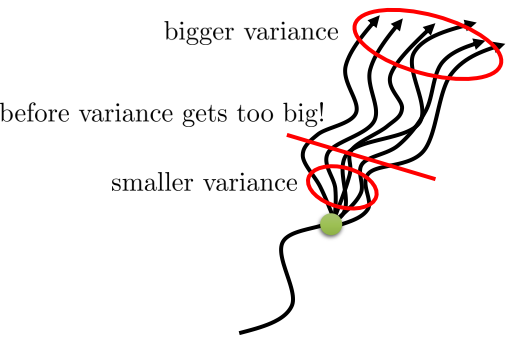$\frac{1}{2} G^2 + \frac{1}{2} G^4$

$G_t^\lambda=(1-\lambda)\sum_{n=1}^\infty \lambda^{n-1}G_t^{(n)} \\ V(S_t) \leftarrow V(S_t) + \alpha (G_t^\lambda-V(S_t))$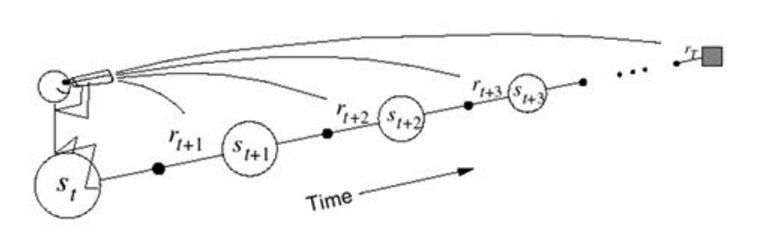$E_0(s)=0\\ E_{t}(s)=\gamma \lambda E_{t-1}(s) + 1(S_t=s)$$\delta_t = R_{t+1} + \gamma V(S_{t+1}) - V(S_t)\\ V(s) = V(s) + \alpha \delta_tE_t(s)$

backward TD是在当前状态下，首先计算出TD Error $\delta_{t}$，然后将TD Error回传，通知以前别的状态处的v做更新，这个更新结合了传过来的TD error和自己状态的Eligibility Traces。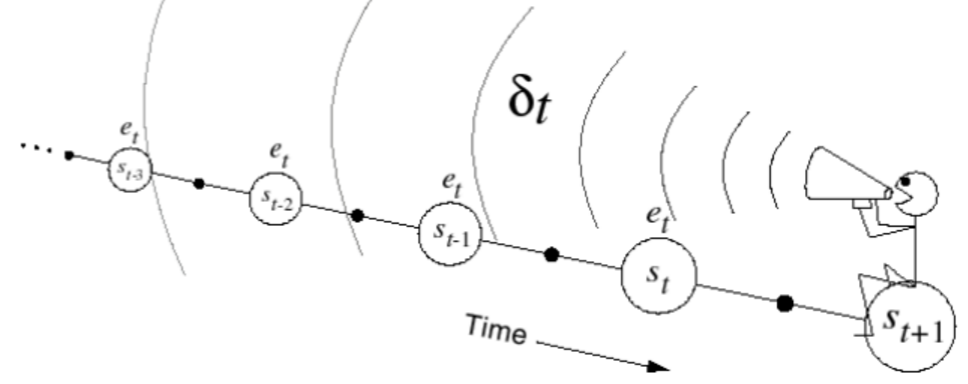#### 6.2.2.4 Relations MC, TD(0) and TD($\lambda$)

TD(0)=TD($\lambda$), $\lambda=0$

$\delta_t = R_{t+1} + \gamma V(S_{t+1}) - V(S_t)\\ V(S_t) = V(S_t) + \alpha \delta_t$

TD($\lambda$)在offline 更新的时候，backward view = forward view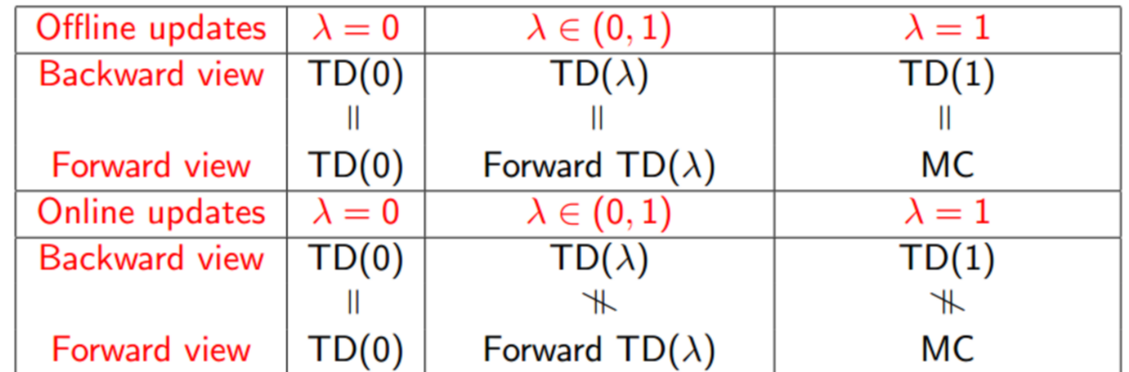#### 6.2.2.5 Off-policy by Importance Sampling

$\frac{\pi_\theta(\tau)}{\pi'(\tau)}=\frac{p(s_1) \prod_{t=1}^T \pi_\theta(a_t\mid s_t)p(s_{t+1}\mid s_t,a_t)}{p(s_1) \prod_{t=1}^T \pi'(a_t\mid s_t)p(s_{t+1}\mid s_t,a_t)} = \prod_{t=1}^T \frac{\pi_\theta(a_t\mid s_t)}{\pi'(a_t\mid s_t)}$

$MC: V(S_t) \leftarrow V(S_t) + \alpha (\frac{\pi_\theta(\tau)}{\pi'(\tau)}G_t-V(S_t))\\ TD: V(S_t) \leftarrow V(S_t) +\alpha (\frac{\pi_\theta(\tau)}{\pi'(\tau)}(R_{t+1} + \gamma V(S_{t+1}) )-V(S_t))$

#### 6.2.2.6 Other Methods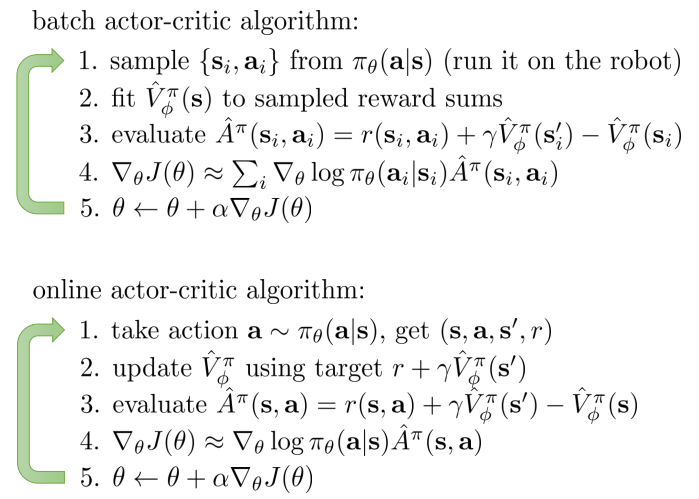$\bigtriangledown_\theta J(\theta)=E_{\tau \sim \pi_\theta(\tau)} [\bigtriangledown_\theta log \pi_\theta(\tau) r(\tau)] \rightarrow \mbox{ REINFORCE algorithm} \\ \bigtriangledown_\theta J(\theta)=E_{\tau \sim \pi_\theta(\tau)} [\bigtriangledown_\theta log \pi_\theta(\tau) Q_w(\tau)] \rightarrow\mbox{ Q Actor-Critic algorithm}\\ \bigtriangledown_\theta J(\theta)=E_{\tau \sim \pi_\theta(\tau)} [\bigtriangledown_\theta log \pi_\theta(\tau) A_w(\tau)]\rightarrow\mbox{ Advantage Actor-Critic algorithm}\\ \bigtriangledown_\theta J(\theta)=E_{\tau \sim \pi_\theta(\tau)} [\bigtriangledown_\theta log \pi_\theta(\tau) \delta ]\rightarrow\mbox{ TD Actor-Critic algorithm}\\ \bigtriangledown_\theta J(\theta)=E_{\tau \sim \pi_\theta(\tau)} [\bigtriangledown_\theta log \pi_\theta(\tau) \delta e]\rightarrow\mbox{ TD(lambda) Actor-Critic algorithm}\\ ...$

$A(s_t,a_t) \approx r(s_t,a_t)+V(s_{t+1}) - V(s_t)$

$\delta=R_{t+1} + \gamma V(s_{t+1})-V(s_t)\\ E_{t}(s)=\lambda E_{t}(s) + \bigtriangledown_\theta log \pi_\theta(s,a)\\ \bigtriangledown_\theta J(\theta)=E_{\tau \sim \pi_\theta(\tau)} [\bigtriangledown_\theta log \pi_\theta(\tau) \delta E]$

# 7. Extracting A Policy From Value Function

$\pi'(a_t\mid s_t)=\left\{\begin{matrix} 1 \mbox{ if } a_t=\arg\max_{a} A^\pi(s_t, a) \\ 0 \mbox{ otherwise} \end{matrix}\right.$

## 7.1 Maximum with known model

$A^\pi(s,a)=Q^\pi(s,a)-V^\pi(s)=r(s,a)+\gamma E_{s' \sim P(s'\mid s,a)}[V^\pi(s')]-V^\pi(s)$

### 7.1.1 Policy Iteration

Policy Iteration是上面问题的一种经典求解算法。具体的方法是使用$\pi’(s)=argmax_{a \in A} q_{\pi}(s,a)$，这样做是去提升，在任意状态s下的决策动作都能有提升。即在当前状态下，贪心的按照能最大化value的动作来走：

$q_\pi(s,\pi'(s)) = max_{a \in A} q_\pi (s,a) \ge q_\pi(s, \pi(s)) = v_\pi(s)$

$v_\pi(s) \leq q_\pi(s, \pi'(s)) = E_{\pi'}[R_{t+1}+\gamma v_\pi(S_{t+1}) \mid S_t=s] \\ \leq E_{\pi'}[R_{t+1}+\gamma q_\pi(S_{t+1}, \pi'(S_{t+1}))\mid S_t=s]\\ \leq E_{\pi'}[R_{t+1}+\gamma R_{t+2} +\gamma q_\pi(S_{t+2}, \pi'(S_{t+2})) \mid S_t=s]\\ \leq E_{\pi'}[R_{t+1}+\gamma R_{t+2} + ... \mid S_t=s] = v_{\pi'}(s)\\$

$q_\pi(s,\pi'(s)) = max_{a \in A} q_\pi (s,a)= q_\pi(s, \pi(s)) = v_\pi(s)$

$max_{a \in A} q_\pi (s,a)=v_\pi(s)$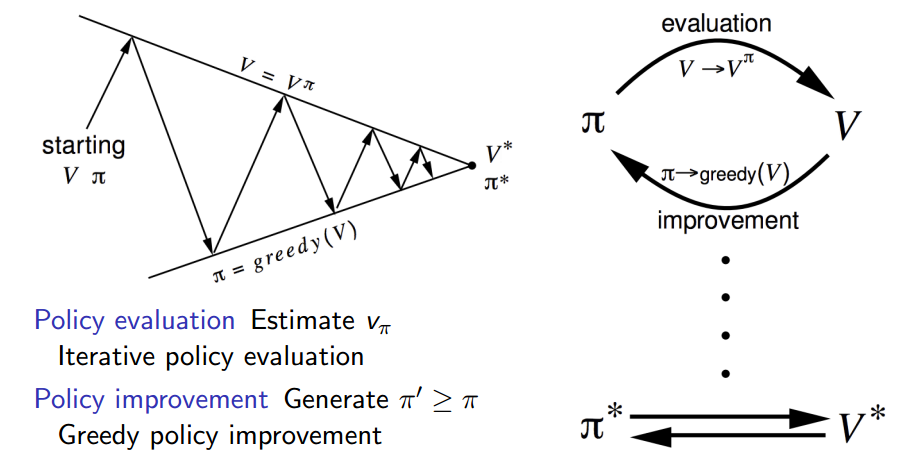1. 左图表示一个迭代之后的结果，也就是迭代之后的state value function。并且每一次迭代之后产生的v都能对应得到一个明确的policy（右图）。
2. 这是policy evaluate的例子，不是iteration，也就是说，没有做policy improve。右图只是画出来的而已。实际上如果要做policy iteration，那么是在左图最后一行的结果上做的improvement（当然实际上不用迭代evaluate到这么多次）。
3. 但事实上，右图所示的最优policy早就出现了，所以可以改进policy iteration
1. 比如，不用等到policy evaluate 收敛之后，再做policy improvement，可以设置阈值或者early stop
2. 当然，也可以每次让evaluate只做一轮迭代（k=1），这就相当于是做了value iteration。那啥是value iteration?

### 7.1.2 Value Iteration

$\pi'(a_t\mid s_t)=\left\{\begin{matrix} 1 \mbox{ if } a_t=\arg\max_{a} A^\pi(s_t, a) \\ 0 \mbox{ otherwise} \end{matrix}\right.\\ A^\pi(s,a)=Q^\pi(s,a)-V^\pi(s)=r(s,a)+\gamma E_{s' \sim P(s'\mid s,a)}[V^\pi(s')]-V^\pi(s)$

1. 一个最优的首个动作a*
2. followed by an optimal policy from successor state S’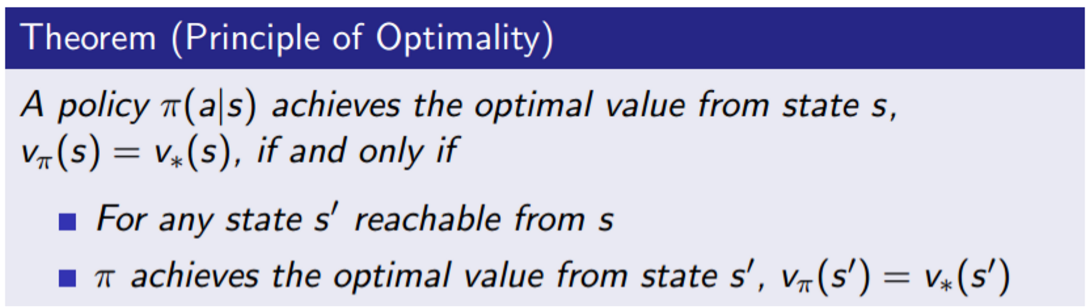value iteration就是利用了这个定理。如果知道了$v_\star(s’)$那么他的前一步最优状态$v_\star(s)$也就可以通过Bellman optimality equation而知道了。

$v_*(s) =\max_{a\in A} \left( R_s^a + \gamma \sum_{s' \in S} P^a_{ss'}v_*(s') \right)$

$v_{k+1}(s) =\max_{a\in A} \left(R_s^a + \gamma \sum_{s' \in S} P^a_{ss'}v_k(s') \right)\\ \mbox{也就是说去迭代：} Q(s,a) = r(s,a) + \gamma E_{P_{ss'}^a}[V(s')]\\ V(s) = max_a Q(s,a)$

$\pi_*(s)=\arg\max_{a \in A} \left( R_{s}^a + \gamma \sum_{s' \in S}P^a_{ss'} v_*(s')\right)$

Policy iteration V.S. Value iterataion

1. value iteration是两步组成：找最优$v_\star$，得到最优$\pi_{\star}$，而policy iteration是一个loop，不断的优化v和$\pi$。这说明了value iteration之所以不迭代，是直接找到了最优的v，它对应的策略也自然是最优的
2. 对于value iteration找最优的v的过程，可以看做是特殊的policy iteration：（k=1的policy evaluate + policy improve的组合），难道只是思想上的相同？？value iteration是用max做一次v的sweep
3. policy iteration的不好之处就是有太多次的policy evaluate，而且每一次都针对所有的状态做更新v(s)（即v_{k+1} -> v_{k}）。所以才会提出value iteration。
4. 两者的复杂度都是很高的！他们都是建立在优化v上，这就意味着每一次迭代有$O(mn^2)$的复杂度。其中m是actions，n是states（给定一个状态，首先遍历m个动作，然后分别能跳转到n个新状态下。这个操作对每个state都得跑一次，所以还得乘一个n，也就是mn^2）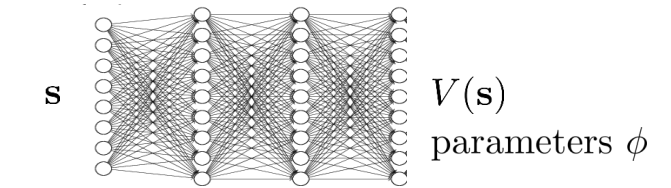$L(\phi)=\frac{1}{2} \mid \mid V_\phi(s) - \max_a Q(s,a) \mid \mid_2^2$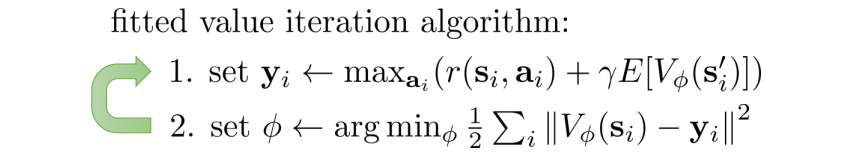## 7.2 Maximum with unkown model

$\pi'(s)=\arg\max_{a} R^a_s + P^a_{ss'} V(s')$

$\pi'(s)=\arg\max_{a} Q(s,a)$

$Q^\pi(s,a) = r(s,a) + \gamma E_{s' \sim P^a_{ss'}} [Q^\pi(s', \pi(s'))]$

$\pi'(a_t\mid s_t)=\left\{\begin{matrix} 1 \mbox{ if } a_t=\arg\max_{a} Q^\pi(s_t, a) \\ 0 \mbox{ otherwise} \end{matrix}\right.$

$\pi(a\mid s)=\left\{\begin{matrix} \epsilon /m + 1 - \epsilon \mbox{ if } a^*=\arg\max_{a \in A} Q(s,a) \\ \epsilon /m \mbox{ otherwise } \end{matrix}\right.$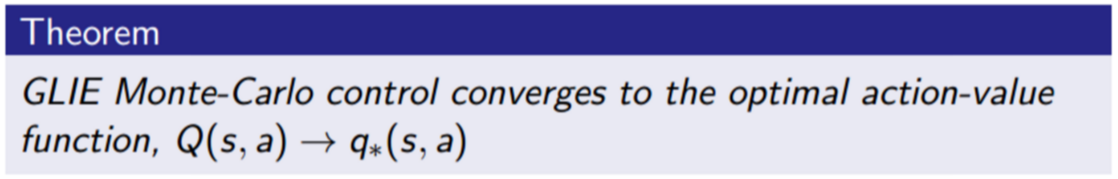### 7.2.1 policy evaluetion on Q with MC

on V

$N(S_t) += 1 \\ V(S_t) \leftarrow V(S_t) + \frac{1}{N(S_{t})} (G_t -V(S_t))$

on Q

$N(S_t, A_t) += 1 \\ Q(S_t, A_t) \leftarrow Q(S_t, A_t) + \frac{1}{N(S_{t}, A_t)} (G_t -Q(S_t, A_t))$

### 7.2.2 policy evaluetion on Q with TD

on V

$V(S_t) \leftarrow V(S_t) +\alpha (R_{t+1} + \gamma V(S_{t+1}) -V(S_t))$

on Q

$Q(S_t, A_t) \leftarrow Q(S_t, A_t) +\alpha (R_{t+1} + \gamma Q(S_{t+1}, A_{t+1}) -Q(S_t, A_t))$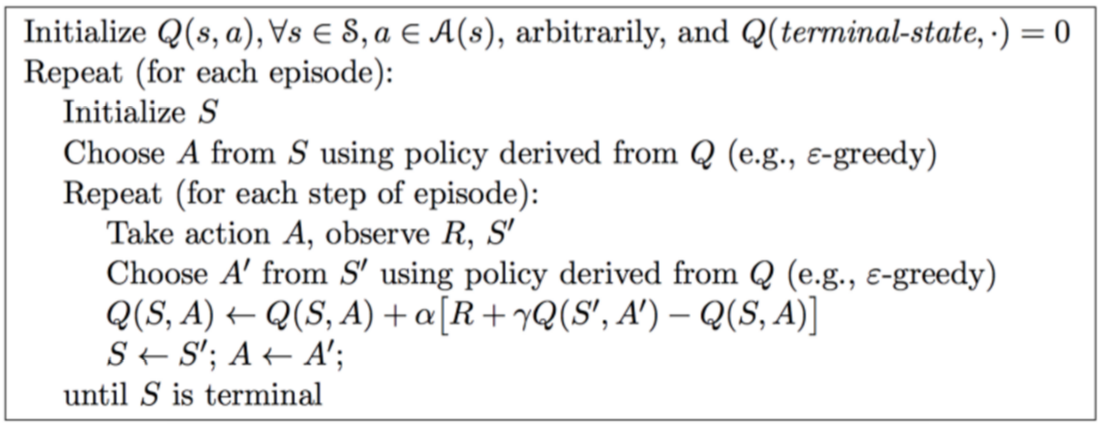### 7.2.3 policy evaluetion on Q with n-step Sarsa & Sarsa($\lambda$)

$q_t^n = R_{t+1} + \gamma R_{t+2} + ... \gamma ^{n-1} R_{t+n} + \gamma^n Q(S_{t+n})\\ Q(S_t, A_t) \leftarrow Q(S_t, A_t) +\alpha (q_t^n -Q(S_t, A_t))$

Forward view Sarsa($\lambda$)

$q_t^\lambda=(1-\lambda)\sum_{n=1}^\infty \lambda^{n-1}q_t^{n} \\ Q(S_t, A_t) \leftarrow Q(S_t, A_t) +\alpha (q_t^\lambda -Q(S_t, A_t))$

Backward view Sarsa($\lambda$) with Eligibility trace

$E_0(s,a)=0\\ E_{t}(s,a)=\gamma \lambda E_{t-1}(s,a) + 1(S_t=s, A_t=a)\\ \delta_t = R_{t+1} + \gamma Q(S_{t+1},A_{t+1}) - Q(S_t,A_t)\\ Q(s,a) = Q(s,a) + \alpha \delta_tE_t(s,a)$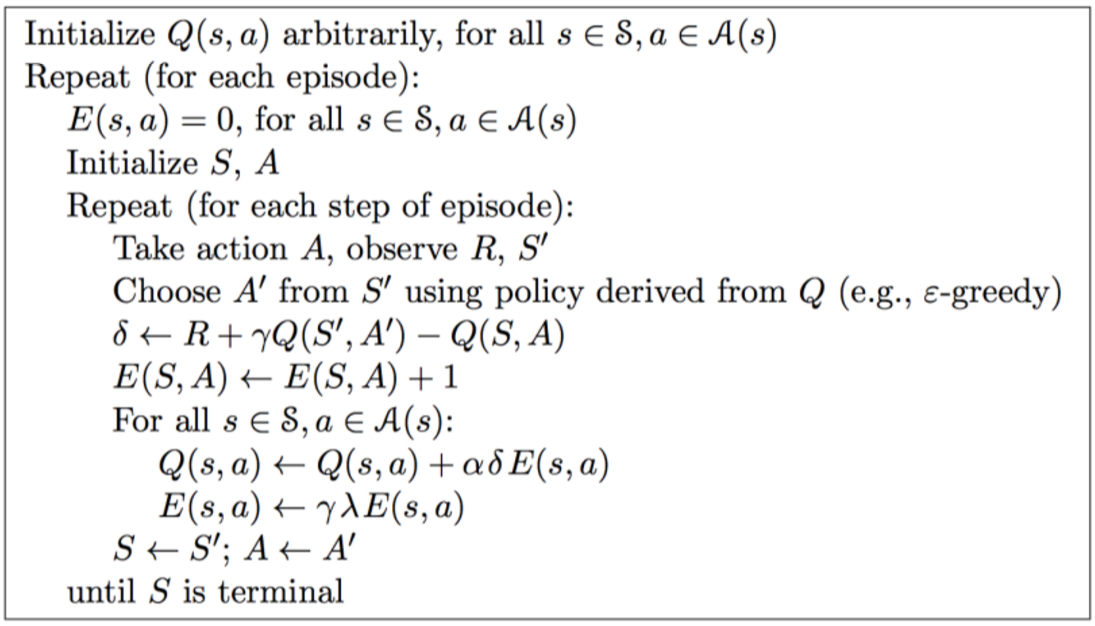### 7.2.3 Off-policy evaluation: Q-Learning$Q(s_t, a_t)=r(s_t,a_t)+\gamma E_{s' \sim P_{s_ts_{t+1}}^{a_t}}[V(s_{t+1})]$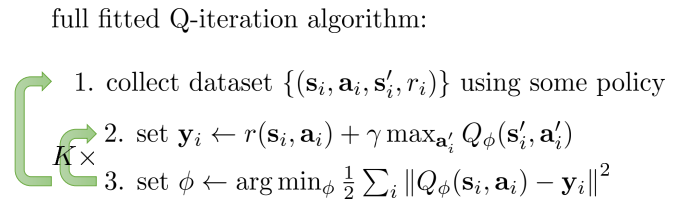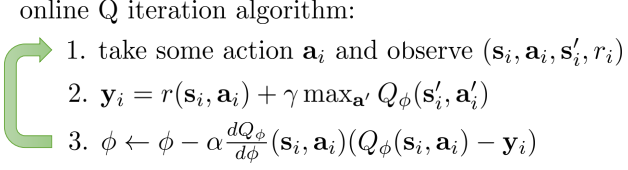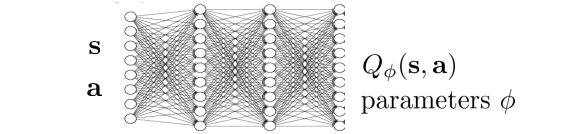$Q-Learing: Q(S_t, A_t) \leftarrow Q(S_t, A_t) +\alpha (R_{t+1} + \gamma \max_{a_{t+1}}Q(S_{t+1}, a_{t+1}) -Q(S_t, A_t))\\ Sarsa: Q(S_t, A_t) \leftarrow Q(S_t, A_t) +\alpha (R_{t+1} + \gamma Q(S_{t+1}, A_{t+1}) -Q(S_t, A_t))$

$q_*(s,a) = R_s^a + \gamma \sum_{s' \in S} P_{ss'}^a \max_a’ q_*(s',a')$

$loss = q_*(s,a) - ( R_s^a + \gamma \max_a’ q_*(s',a'))$

## 7.3 Value function learning theory$\phi \leftarrow \phi - \alpha \frac{dQ_\phi(s_i,a_i)}{d\phi}\left(Q_\phi(s_i,a_i) - [r(s_i,a_i) + \gamma \max_{a'}Q_\phi(s'_i,a'_i)]\right)$

# 8. Advanced Q-Learning with Function Approximation

## 8.1 Function Approximation

### 8.1.1 Why Function Approximation

1. 对于V函数来说，我们需要维护一个从State到V(S)的映射表；对于Q函数来说，我们要维护一个从state和action到Q(s,a)的映射表，甚至对于policy来说，我们还需要一个从state到action的映射表。当state和action的空间很大的时候（Large MDPs），维护这些是很费内存空间的。
2. 当我们使用样本来应对model-free问题的时候，采样样本很可能无法覆盖这个state或者action的空间，那么，这时候学习出来的映射表对于没有出现过的state或者action就无法给出决策。缺乏generalization

$V_\phi(s) \approx V_\pi(s)\\ Q_\phi(s,a) \approx Q_\pi(s,a)\\ \pi_\phi(a\mid s) \approx \pi(a\mid s)$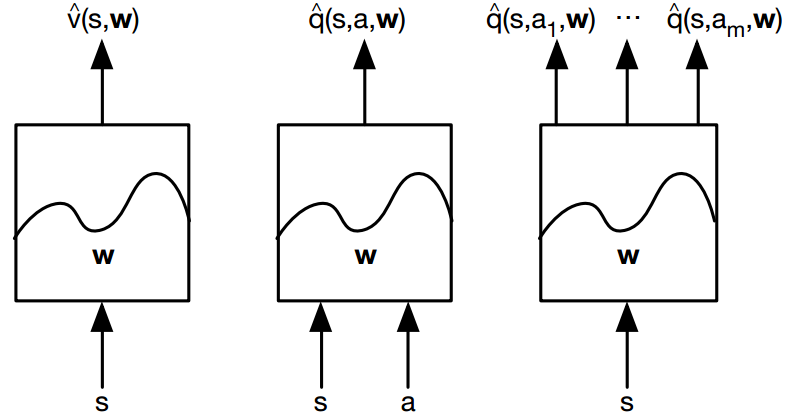### 8.1.2 Policy evaluation with function approximation

$V_\phi(S)=f(S)^T\phi \mbox{ } \mbox{ } \mbox{ } \mbox{ } \mbox{ } Q_\phi(S,A)=f(S,A)^T\phi$

#### 8.1.2.1 Approximating state value function

$\mbox{MC：} V(S_t) \leftarrow V(S_t) +\alpha (G_t -V(S_t))\\ \mbox{TD：} V(S_t) \leftarrow V(S_t) +\alpha (R_{t+1} + \gamma V(S_{t+1}) -V(S_t))\\ \mbox{N-step TD：} V(S_t) \leftarrow V(S_t) +\alpha (G_t^n -V(S_t))\\ \mbox{forward TD(lambda)：} V(S_t) \leftarrow V(S_t) + \alpha (G_t^\lambda-V(S_t))\\ \mbox{backward TD(lambda)：}V(s) = V(s) + \alpha \delta_tE_t(s)\\ E_{t}(s)=\gamma \lambda E_{t-1}(s) + 1(S_t=s)\\ \delta_t = R_{t+1} + \gamma V(S_{t+1}) - V(S_t)\\$

$J(\phi)=E_\pi\left[ (V_\phi(S) - V(S))^2 \right]$

$\triangledown_\phi J(\phi)=(V_\phi(S)-V(S))f(S)\\ \phi \leftarrow \phi - \alpha \triangledown_\phi J(\phi)$

$\mbox{MC：}\triangledown_\phi J(\phi)=(V_\phi(S_t)-G_t)f(S_t)\\ \mbox{TD：}\triangledown_\phi J(\phi)=(V_\phi(S_t)-(R_{t+1} + \gamma V_\phi(S_{t+1})))f(S_t)\\ \mbox{Forward TD(lambda)：}\triangledown_\phi J(\phi)=(V_\phi(S_t)-G_t^\lambda)f(S_t)\\ \mbox{Backward TD(lambda)：}\triangledown_\phi J(\phi)=\delta_tE_t\\ \delta_t= V_\phi(S_t)-R_{t+1} + \gamma V_\phi(S_{t+1})\\ E_t=\gamma \lambda E_{t-1}+f(S_t)$

#### 8.1.2.2 Approximating action value function

$J(\phi)=E_\pi\left[ (q_\phi(S,A)-q(S,A))^2 \right]$

$\mbox{MC：}\triangledown_\phi J(\phi)=(q_\phi(S_t,A_t)-G_t)f(S_t,A_t)\\ \mbox{TD：}\triangledown_\phi J(\phi)=(q_\phi(S_t,A_t)-(R_{t+1} + \gamma q_\phi(S_{t+1},A_{t+1})))f(S_t,A_t) \\ \mbox{Forward TD(lambda)：}\triangledown_\phi J(\phi)=(q_\phi(S_t,A_t)-G_t^\lambda)f(S_t,A_t)\\ \mbox{Backward TD(lambda)：}\triangledown_\phi J(\phi)=\delta_tE_t\\ \delta_t= q_\phi(S_t,A_t)-R_{t+1} + \gamma q_\phi(S_{t+1} ,A_{t+1} )\\ E_t=\gamma \lambda E_{t-1}+f(S_t,A_t)$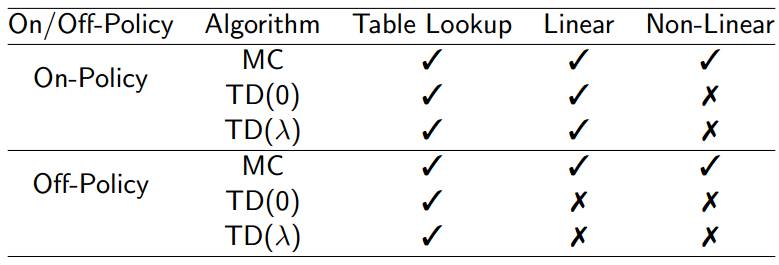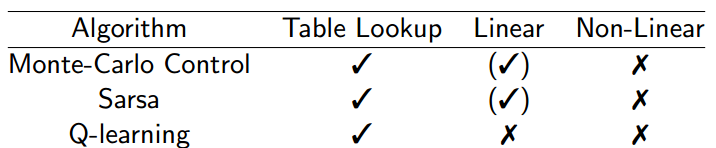## 8.2 Advanced Q-Learning with Deep Network

$\phi \leftarrow \phi - \alpha \frac{dQ_\phi(s_i,a_i)}{d\phi}\left(Q_\phi(s_i,a_i) - [r(s_i,a_i) + \gamma \max_{a'}Q_\phi(s'_i,a'_i)]\right)$### 8.2.1 Experience replay to reduce sample correlation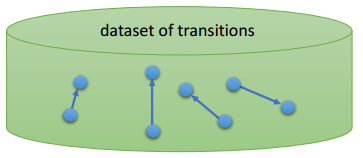1. 给定s，根据当前策略，做出动作a，得到environment的反馈信息
2. 将四元组$(s_{t},a_{t}, s_{t+1},r_{t+1})$放入buffer中（如果buffer满了，就覆盖老的数据）
3. 从buffer中采样一个四元组或者mini-batch的四元组，用这些数据来做当前轮的更新。整个过程就是off-policy的啦### 8.2.2 Fixed Q-target to stabilize gradient

Q-learning效果不稳定的另一个原因就是Q-target老是在变。Fixed Q-target要做的，顾名思义，就是在做更新的时候把Q-target给固定住。为了固定Q-target，这里引入了两套参数（也就对应两个网络）来学习Q。即，原始目标：

$E\left[Q_\phi(s_i,a_i) - [r(s_i,a_i) + \gamma \max_{a'}Q_\phi(s'_i,a'_i)]\right]$

$E\left[Q_{\phi1}(s_i,a_i) - [r(s_i,a_i) + \gamma \max_{a'}Q_{\phi2}(s'_i,a'_i)]\right]$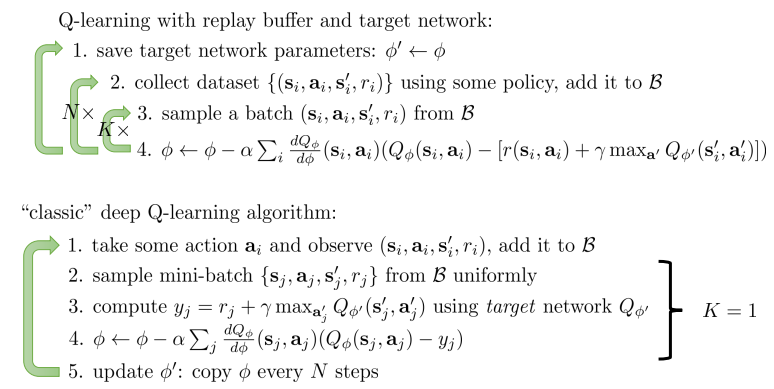### 8.2.3 Polyak-style averaging

1. 第t+1时刻，DQN相当于是使用t时刻的参数拟合Q-target，然后去更新t+1时刻的参数
2. 第t+2时刻，DQN相当于是使用t时刻的参数拟合Q-target，然后去更新t+2时刻的参数
3. 第t+N时刻，DQN相当于是使用t时刻的参数拟合Q-target，然后去更新t+N时刻的参数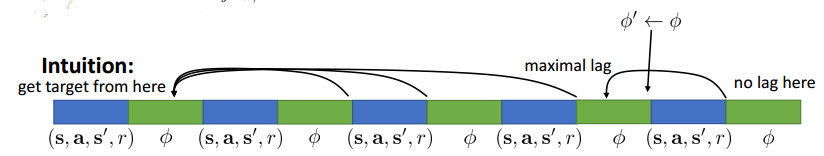$\phi2 = \beta \phi1 + (1-\beta)\phi1$

### 8.2.4 Double Q-Learning to avoid overestimate Q

DQN另一个问题就是，所学习出来的Q和真实的Q值之间的差距太大。一旦Q的值过大，就会使得最后return的值也变大。下图是在不同数据集下所学习出来的效果曲线，其中红色曲线就是DQN的结果，红色横线是真实的结果。为了解决这个问题，double DQN被提了出来，图中，蓝色曲线就是double DQN的结果，蓝色横线是double DQN的真实结果。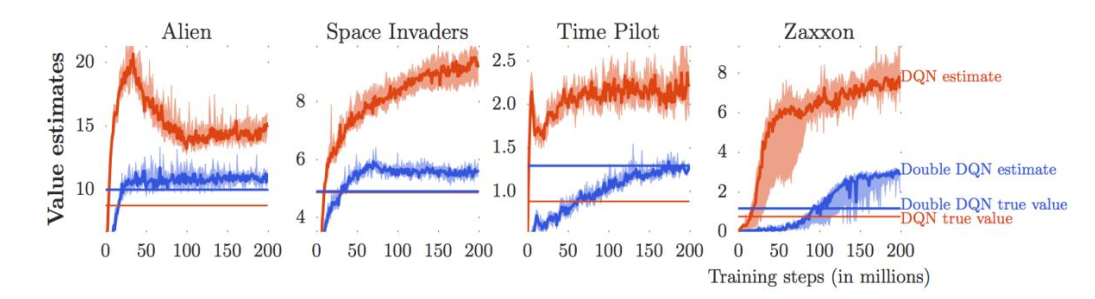DQN（Q-Learning）对Q的估计值过大的问题，Q target中的max造成的。

$\mbox{Q target: }r(s_i,a_i) + \gamma \max_{a'}Q_\phi(s'_i,a'_i)$

$\max_{a'}Q_\phi(s'_i,a'_i)= Q_\phi(s'_i, \arg\max_{a'}Q_\phi(s'_i,a'_i))$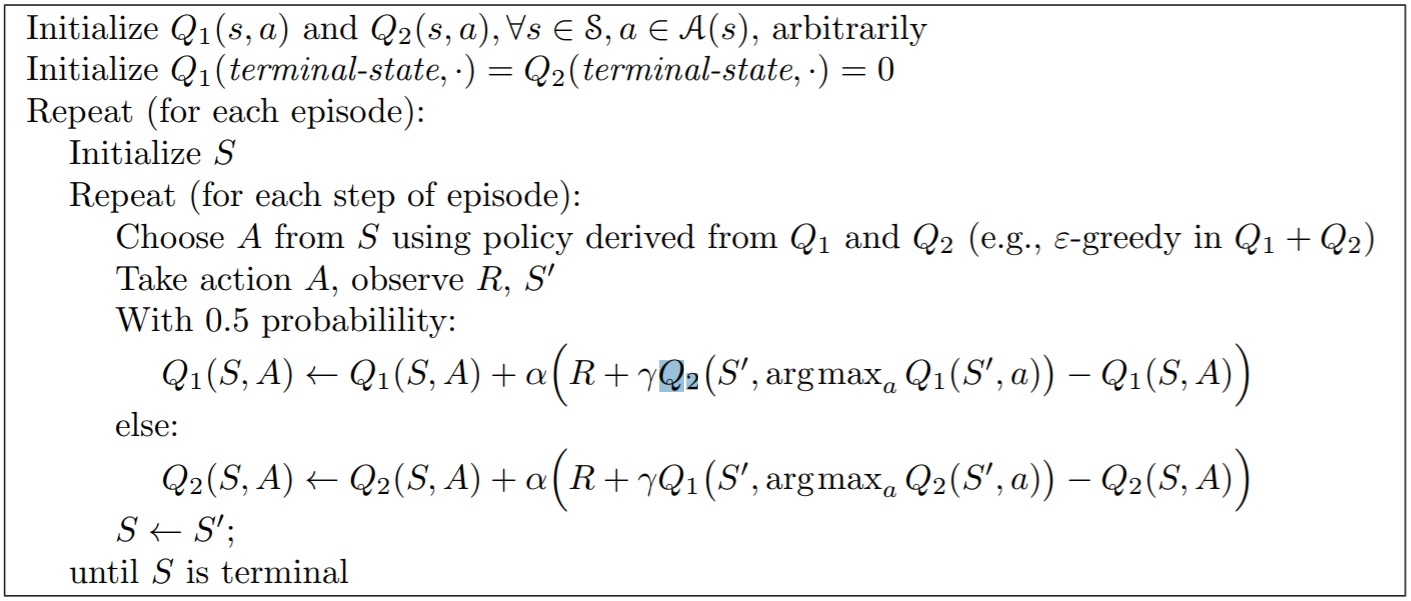### 8.2.6 Continuous actions extension

#### 8.2.6.2 Choose $Q_\phi$ carefully

$Q_\phi(s,a)=-\frac{1}{2}(a - \mu_\phi(s)^T)P_\phi(s)(a-\mu_\phi(s)) + V_\phi(s)$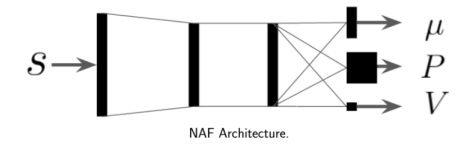#### 8.2.6.3 Learn an approximate maximizer

$\mu_\theta(s) \approx \arg\max_a Q_\phi(s,a)$

$\theta = \arg\max_\theta Q_\phi(s, \mu_\theta(s)) \mbox{ } \rightarrow\mbox{ }\mbox{ } \frac{dQ_\phi}{d\theta}=\frac{da}{d\theta}\frac{Q_\phi}{da}$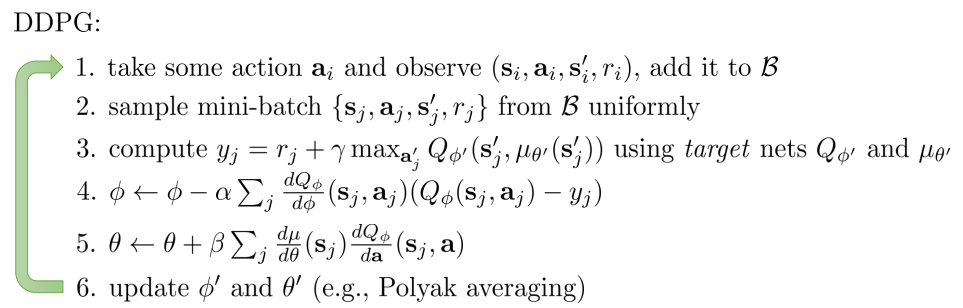# 9. Model-based Planning

planning的方式有很多，policy evaluation是通过动态规划的方式来做的planning，AlphaGo是通过tree search的方式来做的planning。需要注意的是，在做planning的时候，不一定要和environment做交互。如果每个决策都和environment交互，就对应下面左图的closed loop。但是在给定dynamics之后，当environment告诉我们所处的状态，我们就可以做出一个动作序列（因为我已经知道，如果第t时刻我做a，我会被转到s下，那么我就可以直接估计第t+1时刻的动作了，以此类推），不再进行交互了，对于右图的open-loop。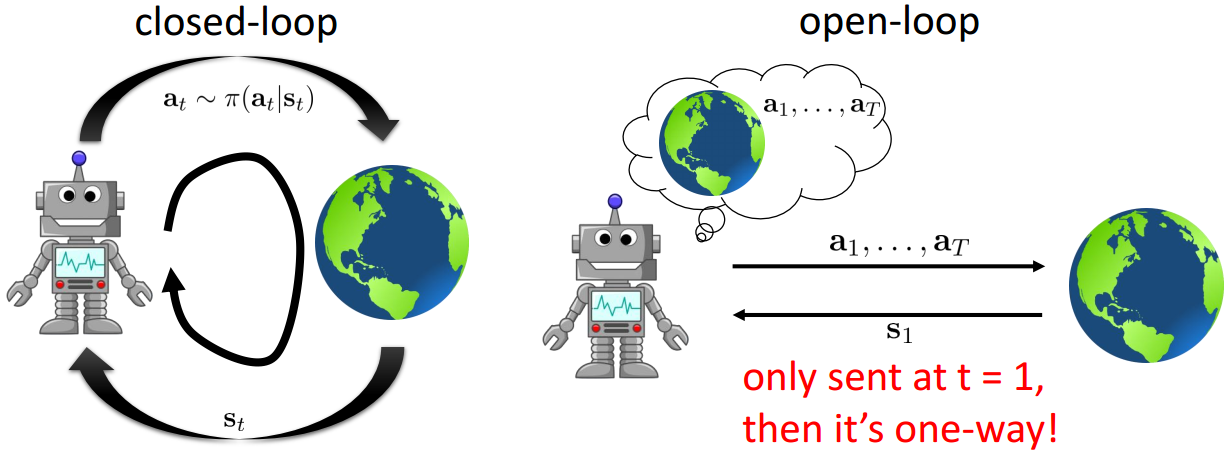$\pi=\arg\max_\pi E_{\tau \sim p(\tau)}\left[ \sum_t \gamma^{t-1} r(s_t,a_t) \right]$

$a_1,...,a_T=\arg\max_{a_1,...,a_T} E_{\tau \sim p(\tau)}\left[ \sum_t \gamma^{t-1} r(s_t,a_t) | a_1,...,a_T\right]$

## 9.1 Stochastic optimization

$a_1,...,a_T=\arg\max_{a_1,...,a_T}J( a_1,...,a_T)$

## 9.2 Monte Carlo tree search (MCTS)

MCTS是在AlphaGo里面用到的方法，他是一种基于树形结构的前向搜索算法，树的节点表示状态。这句话的意思就是，给定当前状态$S_{t}$，以$S_{t}$为根节点建立一棵树向下展开，通过遍历动作空间的取值，跳转到新的状态，依次类推。MCTS并不会就MDP中所有可能的状态都建立成一个大树，而是仅仅为当前时刻下的状态建立一个“子树”。对于子树中的每一个节点（状态），MCTS都会去评估他的value function，以便于在当前时刻下的状态$S_{t}$做出决策动作。当然这颗子树也不会一直展开下去，展开到一定程度之后，将停止展开。对于非叶子节点，在子树展开的过程中就在不断的评估他们的value function，这个展开子树的策略叫做tree policy，而对叶子节点进行评估的policy叫做default policy，一般default policy是一个random policy，也就是下图中的波浪线部分。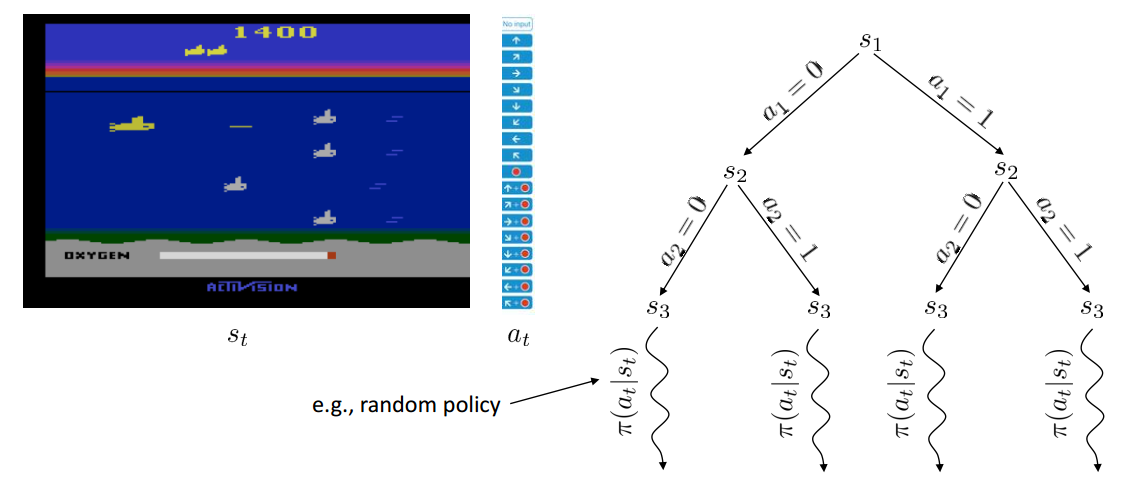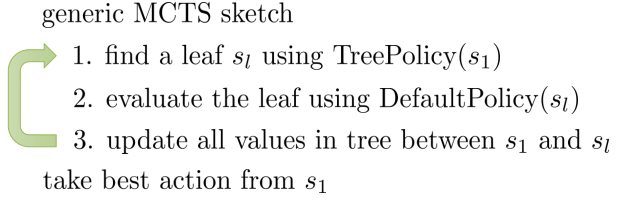Tree policy并不是直接就遍历整个action space了，而是1）首先选择一个没有选过的action；2）如果action都被访问过了，那么就选择一个最好的action走。这里“最好的action”是指按照这个action，会走到一个得分最高的state上。这里state的得分计算公式是一个Upper Confidence Bounds（UCB）。它长成下面这个样子。其中$Fa(s)$表示节点s的父节点，$N(s)$表示节点s被访问过的次数，$Q(s)$，对于叶子节点来说，是它的return（是MC采样得到的？），对于非叶子来说，表示所以孩子节点的Q的和

$Score(s)=\frac{Q(s)}{N(s)} + 2C \sqrt{\frac{2 ln N(Fa(s))}{N(s)}}$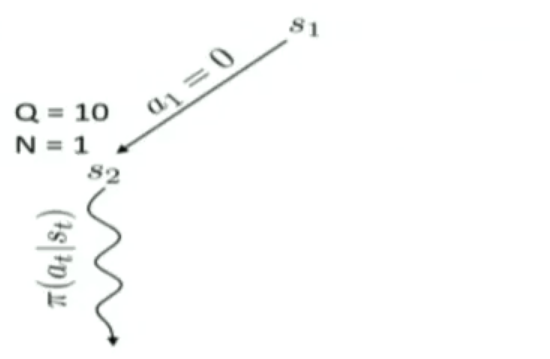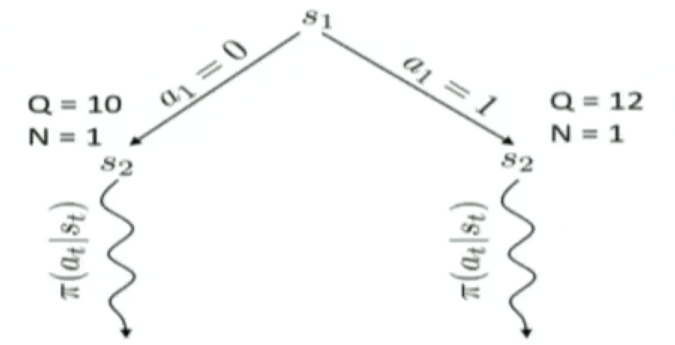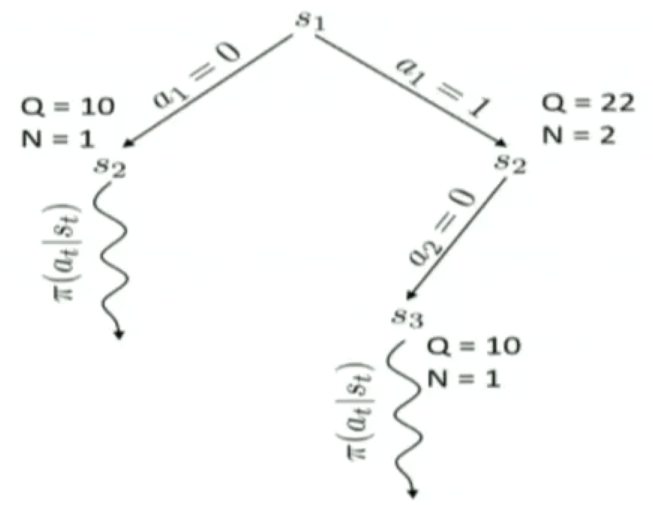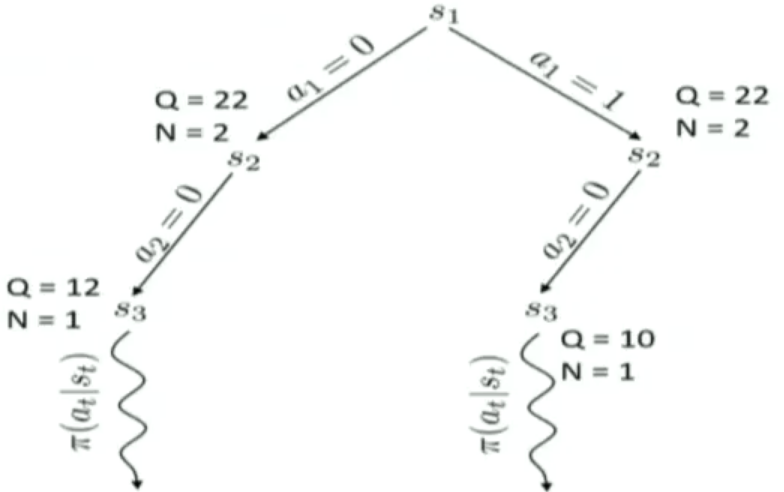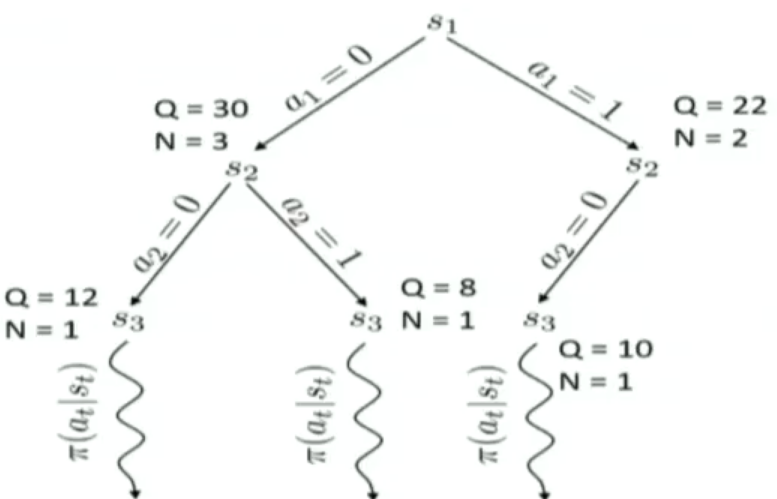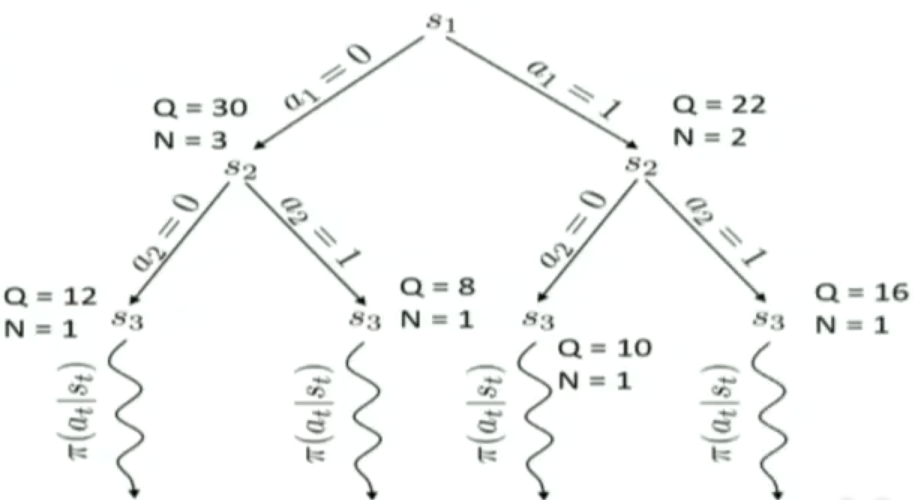## 9.3 Trajectory optimization

### 9.3.1 Linear dynamics case

$\min_{a_1,... ,a_T} \sum_{t=1}^T c(s_t,a_t) \mbox{ s.t. } s_t=f(s_{t-1},a_{t-1})\\ \min_{a_1,... ,a_T} c(s_1,a_1) + c(f(s_1,a_1),a_2)+...+c(f(f(...)),a_T)$

$f(s_t,a_t)=F_t\begin{bmatrix} s_t \\ a_t \end{bmatrix} + f_t\\ c(s_t,a_t)=\frac{1}{2}\begin{bmatrix} s_t \\ a_t \end{bmatrix} ^T C_t \begin{bmatrix} s_t \\ a_t \end{bmatrix} +\begin{bmatrix} s_t \\ a_t \end{bmatrix} ^T c_t$

$a_T=K_Ts_T+k_T\\ K_T=-C^{-1}_{a_T,a_T}C_{a_T,s_T}\\ k_T=-C^{-1}_{a_T,a_T}c_{a_T}\\ C_T=\begin{bmatrix} C_{s_T,s_T} & C_{s_T,a_T} \\ C_{a_T,s_T} & C_{a_T,a_T} \end{bmatrix}\\ c_T=\begin{bmatrix} c_{s_T} \\ c_{a_T} \end{bmatrix}$

$a_{T}$可以用$s_{T}$完全表示，那么可以吧$a_{T}$带回到优化目标的最后一项中，然后，最后一项可以写成：

$V(s_T)=\frac{1}{2}s_T^TV_Ts_T+s_T^Tv_T\\ V_T=C_{s_T,s_T}+C_{s_T,a_T}K_T+K^T_TC_{a_T,s_T}+K^T_TC_{a_T,a_T}K_T\\ v_T=c_{s_T} + C_{s_T,a_T}k_T+K^T_TC_{a_T}+K^T_TC_{a_T,a_T}k_T$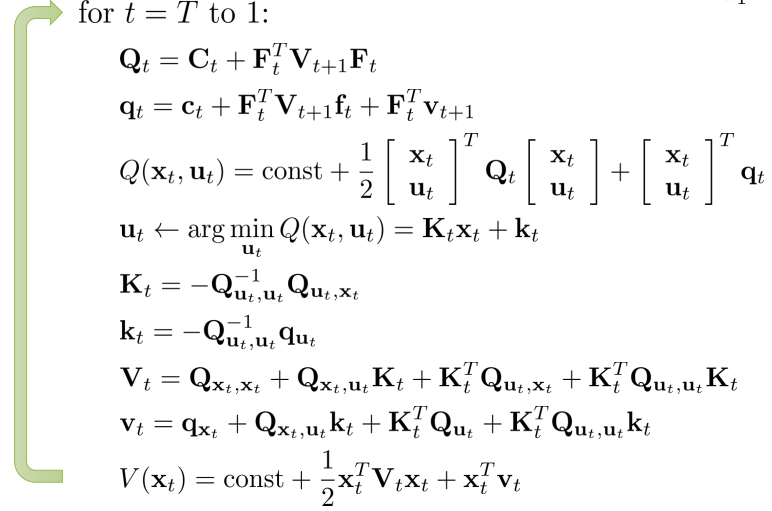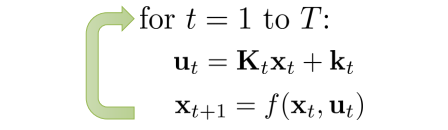### 9.3.2 Non-linear dynamics case

LQR是假设了dynamics是线性的情况，实际上dynamics很可能是非线性的。这就要用到iterative LQR 算法了，他是吧非线性的问题用local linear的方式来近似。近似的方式就是用泰勒展开。对dynamics用泰勒一阶展开成线性的，对cost用二阶展开成quatratic的

$f(s_t,a_t)\approx f(\widehat{s}_t, \widehat{a}_t)+\triangledown_{s_t,a_t}f(\widehat{s}_t, \widehat{a}_t)\begin{bmatrix} s_t-\widehat{s}_t \\ a_t-\widehat{a}_t \end{bmatrix}\\ c(s_t,a_t)\approx c(\widehat{s}_t, \widehat{a}_t)+\triangledown_{s_t,a_t}c(\widehat{s}_t, \widehat{a}_t)\begin{bmatrix} s_t-\widehat{s}_t \\ a_t-\widehat{a}_t \end{bmatrix} + \frac{1}{2}\begin{bmatrix} s_t-\widehat{s}_t \\ a_t-\widehat{a}_t \end{bmatrix}^T\triangledown_{s_t,a_t}^2c(\widehat{s}_t, \widehat{a}_t) \begin{bmatrix} s_t-\widehat{s}_t \\ a_t-\widehat{a}_t \end{bmatrix}$